# Aspect

This tool calculates slope aspect (i.e. slope orientation in degrees clockwise from north) for each grid cell in an input digital elevation model (DEM). The user must specify the name of the input DEM (`--dem`) and the output raster image. The Z conversion factor is only important when the vertical and horizontal units are not the same in the DEM. When this is the case, the algorithm will multiply each elevation in the DEM by the Z conversion factor. If the DEM is in the geographic coordinate system (latitude and longitude), the following equation is used:

zfactor = 1.0 / (111320.0 x cos(mid_lat))

where `mid_lat` is the latitude of the centre of the raster, in radians.

The tool uses Horn's (1981) 3rd-order finite difference method to estimate slope. Given the following clock-type grid cell numbering scheme (Gallant and Wilson, 2000),

| 7 | 8 | 1 |
| 6 | 9 | 2 |
| 5 | 4 | 3 |

aspect = 180 - arctan(fy / fx) + 90(fx / |fx|)

where,

fx = (z3 - z5 + 2(z2 - z6) + z1 - z7) / 8 * Δx

and,

fy = (z7 - z5 + 2(z8 - z4) + z1 - z3) / 8 * Δy

Δx and Δy are the grid resolutions in the x and y direction respectively

Reference:

Gallant, J. C., and J. P. Wilson, 2000, Primary topographic attributes, in Terrain Analysis: Principles and Applications, edited by J. P. Wilson and J. C. Gallant pp. 51-86, John Wiley, Hoboken, N.J.

Parameters:

FlagDescription
-i, --demInput raster DEM file
-o, --outputOutput raster file
--zfactorOptional multiplier for when the vertical and horizontal units are not the same

Python function:

``````wbt.aspect(
dem,
output,
zfactor=None,
callback=default_callback
)
``````

Command-line Interface:

``````>>./whitebox_tools -r=Aspect -v --wd="/path/to/data/" ^
--dem=DEM.tif -o=output.tif
``````

Source code on GitHub

Author: Dr. John Lindsay

Created: 22/06/2017

# AssessRoute

This tool assesses the variability in slope, elevation, and visibility along a line vector, which may be a footpath, road, river or any other route. The user must specify the name of the input line vector (`--routes`), the input raster digital elevation model file (`--dem`), and the output line vector (`--output`). The algorithm initially splits the input line vector in equal-length segments (`--length`). For each line segment, the tool then calculates the average slope (AVG_SLOPE), minimum and maximum elevations (MIN_ELEV, MAX_ELEV), the elevation range or relief (RELIEF), the path sinuosity (SINUOSITY), the number of changes in slope direction or breaks-in-slope (CHG_IN_SLP), and the maximum visibility (VISIBILITY). Each of these metrics are output to the attribute table of the output vector, along with the feature identifier (FID); any attributes associated with the input parent feature will also be copied into the output table. Slope and elevation metrics are measured along the 2D path based on the elevations of each of the row and column intersection points of the raster with the path, estimated from linear-interpolation using the two neighbouring elevations on either side of the path. Sinuosity is calculated as the ratio of the along-surface (i.e. 3D) path length, divided by the 3D distance between the start and end points of the segment. CHG_IN_SLP can be thought of as a crude measure of path roughness, although this will be very sensitive to the quality of the DEM. The visibility metric is based on the Yokoyama et al. (2002) Openness index, which calculates the average horizon angle in the eight cardal directions to a maximum search distance (`--dist`), measured in grid cells.

Note that the input DEM must be in a projected coordinate system. The DEM and the input routes vector must be also share the same coordinate system. This tool also works best when the input DEM is of high quality and fine spatial resolution, such as those derived from LiDAR data sets.

Maximum segment visibility: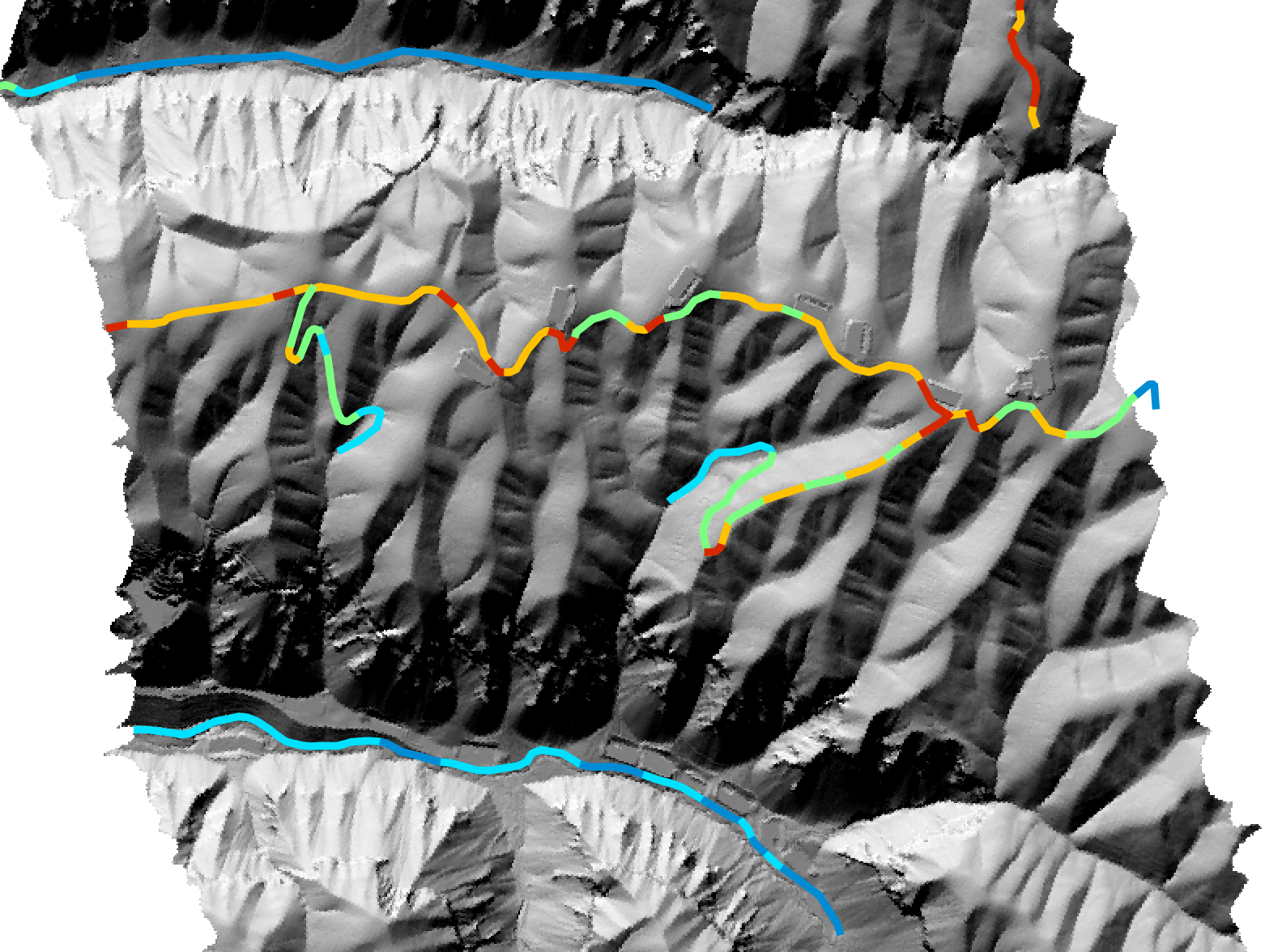Average segment slope: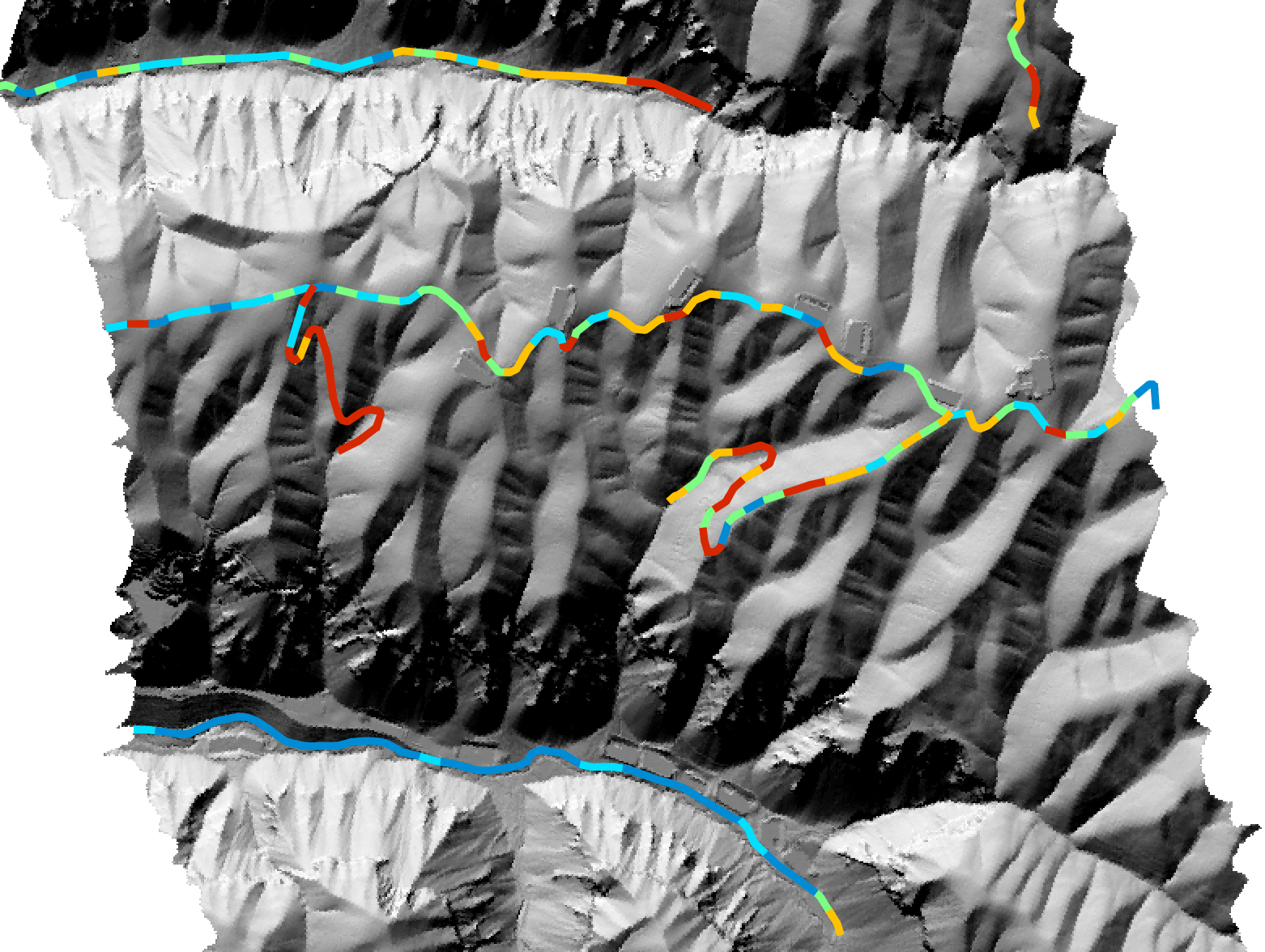Parameters:

FlagDescription
--routesName of the input routes vector file
--demName of the input DEM raster file
-o, --outputName of the output lines shapefile
--lengthMaximum segment length (m)
--distSearch distance, in grid cells, used in visibility analysis

Python function:

``````wbt.assess_route(
routes,
dem,
output,
length="",
dist=20,
callback=default_callback
)
``````

Command-line Interface:

``````>> ./whitebox_tools -r=AssessRoute --routes=footpath.shp ^
--dem=DEM.tif -o=assessedRoutes.shp --length=50.0 --dist=200
``````

Source code is unavailable due to proprietary license.

Author: Whitebox Geospatial Inc. (c)

Created: 16/05/2021

# AverageNormalVectorAngularDeviation

This tool characterizes the spatial distribution of the average normal vector angular deviation, a measure of surface roughness. Working in the field of 3D printing, Ko et al. (2016) defined a measure of surface roughness based on quantifying the angular deviations in the direction of the normal vector of a real surface from its ideal (i.e. smoothed) form. This measure of surface complexity is therefore in units of degrees. Specifically, roughness is defined in this study as the neighborhood-averaged difference in the normal vectors of the original DEM and a smoothed DEM surface. Smoothed surfaces are derived by applying a Gaussian blur of the same size as the neighborhood (`--filter`).

The MultiscaleRoughness tool calculates the same measure of surface roughness, except that it is designed to work with multiple spatial scales.

Reference:

Ko, M., Kang, H., ulrim Kim, J., Lee, Y., & Hwang, J. E. (2016, July). How to measure quality of affordable 3D printing: Cultivating quantitative index in the user community. In International Conference on Human-Computer Interaction (pp. 116-121). Springer, Cham.

Lindsay, J. B., & Newman, D. R. (2018). Hyper-scale analysis of surface roughness. PeerJ Preprints, 6, e27110v1.

Parameters:

FlagDescription
-i, --demInput raster DEM file
--outputOutput raster file
--filterSize of the filter kernel

Python function:

``````wbt.average_normal_vector_angular_deviation(
dem,
output,
filter=11,
callback=default_callback
)
``````

Command-line Interface:

``````>>./whitebox_tools -r=AverageNormalVectorAngularDeviation -v ^
--wd="/path/to/data/" --dem=DEM.tif --out_mag=roughness_mag.tif ^
--out_scale=roughness_scale.tif --min_scale=1 --max_scale=1000 ^
--step=5
``````

Source code on GitHub

Author: Dr. John Lindsay

Created: 26/01/2019

# CircularVarianceOfAspect

This tool can be used to calculate the circular variance (i.e. one minus the mean resultant length) of aspect for an input digital elevation model (DEM). This is a measure of how variable slope aspect is within a local neighbourhood of a specified size (`--filter`). CircularVarianceOfAspect is therefore a measure of surface shape complexity, or texture. It will take a value of 0.0 for smooth sites and near 1.0 in areas of high surface roughness or complex topography.

The local neighbourhood size (`--filter`) must be any odd integer equal to or greater than three. Grohmann et al. (2010) found that vector dispersion, a related measure of angular variance, increases monotonically with scale. This is the result of the angular dispersion measure integrating (accumulating) all of the surface variance of smaller scales up to the test scale. A more interesting scale relation can therefore be estimated by isolating the amount of surface complexity associated with specific scale ranges. That is, at large spatial scales, the metric should reflect the texture of large-scale landforms rather than the accumulated complexity at all smaller scales, including microtopographic roughness. As such, this tool normalizes the surface complexity of scales that are smaller than the filter size by applying Gaussian blur (with a standard deviation of one-third the filter size) to the DEM prior to calculating CircularVarianceOfAspect. In this way, the resulting distribution is able to isolate and highlight the surface shape complexity associated with landscape features of a similar scale to that of the filter size.

This tool makes extensive use of integral images (i.e. summed-area tables) and parallel processing to ensure computational efficiency. It may, however, require substantial memory resources when applied to larger DEMs.

References:

Grohmann, C. H., Smith, M. J., & Riccomini, C. (2010). Multiscale analysis of topographic surface roughness in the Midland Valley, Scotland. IEEE Transactions on Geoscience and Remote Sensing, 49(4), 1200-1213.

Parameters:

FlagDescription
-i, --demInput raster DEM file
--outputOutput raster file
--filterSize of the filter kernel

Python function:

``````wbt.circular_variance_of_aspect(
dem,
output,
filter=11,
callback=default_callback
)
``````

Command-line Interface:

``````>>./whitebox_tools -r=CircularVarianceOfAspect -v ^
--wd="/path/to/data/" --dem=DEM.tif --out_mag=roughness_mag.tif ^
--out_scale=roughness_scale.tif --min_scale=1 --max_scale=1000 ^
--step=5
``````

Source code on GitHub

Author: Dr. John Lindsay

Created: 26/01/2019

# ContoursFromPoints

This tool creates a contour coverage from a set of input points (`--input`). The user must specify the contour interval (`--interval`) and optionally, the base contour value (`--base`). The degree to which contours are smoothed is controlled by the Smoothing Filter Size parameter (`--smooth`). This value, which determines the size of a mean filter applied to the x-y position of vertices in each contour, should be an odd integer value, e.g. 3, 5, 7, 9, 11, etc. Larger values will result in smoother contour lines.

Parameters:

FlagDescription
-i, --inputInput vector points file
--fieldInput field name in attribute table
--use_zUse the 'z' dimension of the Shapefile's geometry instead of an attribute field?
-o, --outputOutput vector lines file
--max_triangle_edge_lengthOptional maximum triangle edge length; triangles larger than this size will not be gridded
--intervalContour interval
--baseBase contour height
--smoothSmoothing filter size (in num. points), e.g. 3, 5, 7, 9, 11

Python function:

``````wbt.contours_from_points(
i,
output,
field=None,
use_z=False,
max_triangle_edge_length=None,
interval=10.0,
base=0.0,
smooth=5,
callback=default_callback
)
``````

Command-line Interface:

``````>>./whitebox_tools -r=ContoursFromPoints -v ^
--wd="/path/to/data/" -i=points.shp --field=HEIGHT ^
-o=contours.shp --max_triangle_edge_length=100.0 ^
--interval=100.0 --base=0.0 --smooth=11
``````

Source code on GitHub

Author: Dr. John Lindsay

Created: 26/04/2020

# ContoursFromRaster

This tool can be used to create a vector contour coverage from an input raster surface model (`--input`), such as a digital elevation model (DEM). The user must specify the contour interval (`--interval`) and optionally, the base contour value (`--base`). The degree to which contours are smoothed is controlled by the Smoothing Filter Size parameter (`--smooth`). This value, which determines the size of a mean filter applied to the x-y position of vertices in each contour, should be an odd integer value, e.g. 3, 5, 7, 9, 11, etc. Larger values will result in smoother contour lines. The tolerance parameter (`--tolerance`) controls the amount of line generalization. That is, vertices in a contour line will be selectively removed from the line if they do not result in an angular deflection in the line's path of at least this threshold value. Increasing this value can significantly decrease the size of the output contour vector file, at the cost of generating straighter contour line segments.

Parameters:

FlagDescription
-i, --inputInput surface raster file
-o, --outputOutput vector contour file
--intervalContour interval
--baseBase contour height
--smoothSmoothing filter size (in num. points), e.g. 3, 5, 7, 9, 11
--toleranceTolerance factor, in degrees (0-45); determines generalization level

Python function:

``````wbt.contours_from_raster(
i,
output,
interval=10.0,
base=0.0,
smooth=9,
tolerance=10.0,
callback=default_callback
)
``````

Command-line Interface:

``````>>./whitebox_tools -r=ContoursFromRaster -v ^
--wd="/path/to/data/" --input=DEM.tif -o=contours.shp ^
--interval=100.0 --base=0.0 --smooth=11 --tolerance=20.0
``````

Source code on GitHub

Author: Dr. John Lindsay

Created: 22/02/2020

# DevFromMeanElev

This tool can be used to calculate the difference between the elevation of each grid cell and the mean elevation of the centering local neighbourhood, normalized by standard deviation. Therefore, this index of topographic residual is essentially equivalent to a local z-score. This attribute measures the relative topographic position as a fraction of local relief, and so is normalized to the local surface roughness. DevFromMeanElev utilizes an integral image approach (Crow, 1984) to ensure highly efficient filtering that is invariant with filter size.

The user must specify the name (`--dem`) of the input digital elevation model (DEM), the name of the output file (`--output`), and the size of the neighbourhood in the x and y directions (`--filterx` and `--filtery`), measured in grid size.

While DevFromMeanElev calculates the deviation from mean elevation (DEV) at a single, user-defined scale, the MaxElevationDeviation tool can be used to output the per-pixel maximum DEV value across a range of input scales.

Parameters:

FlagDescription
-i, --input, --demInput raster DEM file
-o, --outputOutput raster file
--filterxSize of the filter kernel in the x-direction
--filterySize of the filter kernel in the y-direction

Python function:

``````wbt.dev_from_mean_elev(
dem,
output,
filterx=11,
filtery=11,
callback=default_callback
)
``````

Command-line Interface:

``````>>./whitebox_tools -r=DevFromMeanElev -v ^
--wd="/path/to/data/" --dem=DEM.tif -o=output.tif ^
--filter=25
``````

Source code on GitHub

Author: Dr. John Lindsay

Created: 21/06/2017

# DiffFromMeanElev

This tool can be used to calculate the difference between the elevation of each grid cell and the mean elevation of the centering local neighbourhood. This is similar to what a high-pass filter calculates for imagery data, but is intended to work with DEM data instead. This attribute measures the relative topographic position. DiffFromMeanElev utilizes an integral image approach (Crow, 1984) to ensure highly efficient filtering that is invariant with filter size.

The user must specify the name (`--dem`) of the input digital elevation model (DEM), the name of the output file (`--output`), and the size of the neighbourhood in the x and y directions (`--filterx` and `--filtery`), measured in grid size.

While DevFromMeanElev calculates the DIFF at a single, user-defined scale, the MaxDifferenceFromMean tool can be used to output the per-pixel maximum DIFF value across a range of input scales.

Parameters:

FlagDescription
-i, --input, --demInput raster DEM file
-o, --outputOutput raster file
--filterxSize of the filter kernel in the x-direction
--filterySize of the filter kernel in the y-direction

Python function:

``````wbt.diff_from_mean_elev(
dem,
output,
filterx=11,
filtery=11,
callback=default_callback
)
``````

Command-line Interface:

``````>>./whitebox_tools -r=DiffFromMeanElev -v ^
--wd="/path/to/data/" --dem=DEM.tif -o=output.tif ^
--filter=25
``````

Source code on GitHub

Author: Dr. John Lindsay

Created: 25/06/2017

# DirectionalRelief

This tool calculates the relief for each grid cell in a digital elevation model (DEM) in a specified direction. Directional relief is an index of the degree to which a DEM grid cell is higher or lower than its surroundings. It is calculated by subtracting the elevation of a DEM grid cell from the average elevation of those cells which lie between it and the edge of the DEM in a specified compass direction. Thus, positive values indicate that a grid cell is lower than the average elevation of the grid cells in a specific direction (i.e. relatively sheltered), whereas a negative directional relief indicates that the grid cell is higher (i.e. relatively exposed). The algorithm is based on a modification of the procedure described by Lapen and Martz (1993). The modifications include: (1) the ability to specify any direction between 0-degrees and 360-degrees (`--azimuth`), and (2) the ability to use a distance-limited search (`--max_dist`), such that the ray-tracing procedure terminates before the DEM edge is reached for longer search paths. The algorithm works by tracing a ray from each grid cell in the direction of interest and evaluating the average elevation along the ray. Linear interpolation is used to estimate the elevation of the surface where a ray does not intersect the DEM grid precisely at one of its nodes. The user must specify the name of an input DEM raster file, the output raster name, and a hypothetical wind direction. Furthermore, the user is able to constrain the maximum search distance for the ray tracing. If no maximum search distance is specified, each ray will be traced to the edge of the DEM. The units of the output image are the same as the input DEM.

Ray-tracing is a highly computationally intensive task and therefore this tool may take considerable time to operate for larger sized DEMs. This tool is parallelized to aid with computational efficiency. NoData valued grid cells in the input image will be assigned NoData values in the output image. The output raster is of the float data type and continuous data scale. Directional relief is best displayed using the blue-white-red bipolar palette to distinguish between the positive and negative values that are present in the output.

Reference:

Lapen, D. R., & Martz, L. W. (1993). The measurement of two simple topographic indices of wind sheltering-exposure from raster digital elevation models. Computers & Geosciences, 19(6), 769-779.

Parameters:

FlagDescription
-i, --demInput raster DEM file
-o, --outputOutput raster file
--azimuthWind azimuth in degrees
--max_distOptional maximum search distance (unspecified if none; in xy units)

Python function:

``````wbt.directional_relief(
dem,
output,
azimuth=0.0,
max_dist=None,
callback=default_callback
)
``````

Command-line Interface:

``````>>./whitebox_tools -r=DirectionalRelief -v ^
--wd="/path/to/data/" -i='input.tif' -o=output.tif ^
--azimuth=315.0
``````

Source code on GitHub

Author: Dr. John Lindsay

Created: 07/07/2017

# DownslopeIndex

This tool can be used to calculate the downslope index described by Hjerdt et al. (2004). The downslope index is a measure of the slope gradient between a grid cell and some downslope location (along the flowpath passing through the upslope grid cell) that represents a specified vertical drop (i.e. a potential head drop). The index has been shown to be useful for hydrological, geomorphological, and biogeochemical applications.

The user must specify the name of a digital elevaton model (DEM) raster. This DEM should be have been pre-processed to remove artifact topographic depressions and flat areas. The user must also specify the head potential drop (d), and the output type. The output type can be either '`tangent`', '`degrees`', '`radians`', or '`distance`'. If '`distance`' is selected as the output type, the output grid actually represents the downslope flowpath length required to drop d meters from each grid cell. Linear interpolation is used when the specified drop value is encountered between two adjacent grid cells along a flowpath traverse.

Notice that this algorithm is affected by edge contamination. That is, for some grid cells, the edge of the grid will be encountered along a flowpath traverse before the specified vertical drop occurs. In these cases, the value of the downslope index is approximated by replacing d with the actual elevation drop observed along the flowpath. To avoid this problem, the entire watershed containing an area of interest should be contained in the DEM.

Grid cells containing NoData values in any of the input images are assigned the NoData value in the output raster. The output raster is of the float data type and continuous data scale.

Reference:

Hjerdt, K.N., McDonnell, J.J., Seibert, J. Rodhe, A. (2004) A new topographic index to quantify downslope controls on local drainage, Water Resources Research, 40, W05602, doi:10.1029/2004WR003130.

Parameters:

FlagDescription
-i, --demInput raster DEM file
-o, --outputOutput raster file
--dropVertical drop value (default is 2.0)
--out_typeOutput type, options include 'tangent', 'degrees', 'radians', 'distance' (default is 'tangent')

Python function:

``````wbt.downslope_index(
dem,
output,
drop=2.0,
out_type="tangent",
callback=default_callback
)
``````

Command-line Interface:

``````>>./whitebox_tools -r=DownslopeIndex -v --wd="/path/to/data/" ^
--dem=pointer.tif -o=dsi.tif --drop=5.0 --out_type=distance
``````

Source code on GitHub

Author: Dr. John Lindsay

Created: July 17, 2017

# EdgeDensity

This tool calculates the density of edges, or breaks-in-slope within an input digital elevation model (DEM). A break-in-slope occurs between two neighbouring grid cells if the angular difference between their normal vectors is greater than a user-specified threshold value (`--norm_diff`). EdgeDensity calculates the proportion of edge cells within the neighbouring window, of square filter dimension `--filter`, surrounding each grid cell. Therefore, `EdgeDensity `is a measure of how complex the topographic surface is within a local neighbourhood. It is therefore a measure of topographic texture. It will take a value near 0.0 for smooth sites and 1.0 in areas of high surface roughness or complex topography.

The distribution of EdgeDensity is highly dependent upon the value of the `norm_diff` used in the calculation. This threshold may require experimentation to find an appropriate value and is likely dependent upon the topography and source data. Nonetheless, experience has shown that EdgeDensity provides one of the best measures of surface texture of any of the available roughness tools.

Parameters:

FlagDescription
-i, --demInput raster DEM file
-o, --outputOutput raster file
--filterSize of the filter kernel
--norm_diffMaximum difference in normal vectors, in degrees
--zfactorOptional multiplier for when the vertical and horizontal units are not the same

Python function:

``````wbt.edge_density(
dem,
output,
filter=11,
norm_diff=5.0,
zfactor=None,
callback=default_callback
)
``````

Command-line Interface:

``````>>./whitebox_tools -r=EdgeDensity -v --wd="/path/to/data/" ^
--dem=DEM.tif -o=output.tif --filter=15 --norm_diff=20.0 ^
--num_iter=4
``````

Source code on GitHub

Author: Dr. John Lindsay

Created: 27/01/2019

# ElevAbovePit

This tool will calculate the elevation of each grid cell in a digital elevation model (DEM) above the nearest downslope pit cell or grid edge cell, depending on which is encountered first during the flow-path traverse. The resulting image is therefore a measure of relative landscape position. The user must specify the names of a D8 flow pointer grid, a DEM file, and the output file. The flow pointer grid must be derived using the D8 flow algorithm.

Parameters:

FlagDescription
-i, --demInput raster DEM file
-o, --outputOutput raster file

Python function:

``````wbt.elev_above_pit(
dem,
output,
callback=default_callback
)
``````

Command-line Interface:

``````>>./whitebox_tools -r=ElevAbovePit -v --wd="/path/to/data/" ^
--dem=DEM.tif -o=output.tif
``````

Source code on GitHub

Author: Dr. John Lindsay

Created: 13/07/2017

# ElevPercentile

Elevation percentile (EP) is a measure of local topographic position (LTP). It expresses the vertical position for a digital elevation model (DEM) grid cell (z0) as the percentile of the elevation distribution within the filter window, such that:

EP = counti∈C(zi > z0) x (100 / nC)

where z0 is the elevation of the window's center grid cell, zi is the elevation of cell i contained within the neighboring set C, and nC is the number of grid cells contained within the window.

EP is unsigned and expressed as a percentage, bound between 0% and 100%. Quantile-based estimates (e.g., the median and interquartile range) are often used in nonparametric statistics to provide data variability estimates without assuming the distribution is normal. Thus, EP is largely unaffected by irregularly shaped elevation frequency distributions or by outliers in the DEM, resulting in a highly robust metric of LTP. In fact, elevation distributions within small to medium sized neighborhoods often exhibit skewed, multimodal, and non-Gaussian distributions, where the occurrence of elevation errors can often result in distribution outliers. Thus, based on these statistical characteristics, EP is considered one of the most robust representation of LTP.

The algorithm implemented by this tool uses the relatively efficient running-histogram filtering algorithm of Huang et al. (1979). Because most DEMs contain floating point data, elevation values must be rounded to be binned. The `--sig_digits` parameter is used to determine the level of precision preserved during this binning process. The algorithm is parallelized to further aid with computational efficiency.

Neighbourhood size, or filter size, is specified in the x and y dimensions using the `--filterx` and `--filtery`flags. These dimensions should be odd, positive integer values (e.g. 3, 5, 7, 9, etc.).

References:

Newman, D. R., Lindsay, J. B., and Cockburn, J. M. H. (2018). Evaluating metrics of local topographic position for multiscale geomorphometric analysis. Geomorphology, 312, 40-50.

Huang, T., Yang, G.J.T.G.Y. and Tang, G., 1979. A fast two-dimensional median filtering algorithm. IEEE Transactions on Acoustics, Speech, and Signal Processing, 27(1), pp.13-18.

Parameters:

FlagDescription
-i, --input, --demInput raster DEM file
-o, --outputOutput raster file
--filterxSize of the filter kernel in the x-direction
--filterySize of the filter kernel in the y-direction
--sig_digitsNumber of significant digits

Python function:

``````wbt.elev_percentile(
dem,
output,
filterx=11,
filtery=11,
sig_digits=2,
callback=default_callback
)
``````

Command-line Interface:

``````>>./whitebox_tools -r=ElevPercentile -v --wd="/path/to/data/" ^
--dem=DEM.tif -o=output.tif --filter=25
``````

Source code on GitHub

Author: Dr. John Lindsay

Created: 22/06/2017

# ElevRelativeToMinMax

This tool can be used to express the elevation of a grid cell in a digital elevation model (DEM) as a percentage of the relief between the DEM minimum and maximum values. As such, it provides a basic measure of relative topographic position.

Parameters:

FlagDescription
-i, --demInput raster DEM file
-o, --outputOutput raster file

Python function:

``````wbt.elev_relative_to_min_max(
dem,
output,
callback=default_callback
)
``````

Command-line Interface:

``````>>./whitebox_tools -r=ElevRelativeToMinMax -v ^
--wd="/path/to/data/" --dem=DEM.tif -o=output.tif
``````

Source code on GitHub

Author: Dr. John Lindsay

Created: 12/07/2017

# ElevRelativeToWatershedMinMax

This tool can be used to express the elevation of a grid cell in a digital elevation model (DEM) as a percentage of the relief between the watershed minimum and maximum values. As such, it provides a basic measure of relative topographic position. The user must specify the names of DEM (`--dem`) and watersheds (`--watersheds`) raster files.

Parameters:

FlagDescription
-i, --demInput raster DEM file
--watershedsInput raster watersheds file
-o, --outputOutput raster file

Python function:

``````wbt.elev_relative_to_watershed_min_max(
dem,
watersheds,
output,
callback=default_callback
)
``````

Command-line Interface:

``````>>./whitebox_tools -r=ElevRelativeToWatershedMinMax -v ^
--wd="/path/to/data/" --dem=DEM.tif --watersheds=watershed.tif ^
-o=output.tif
``````

Source code on GitHub

Author: Dr. John Lindsay

Created: 12/07/2017

# EmbankmentMapping

This tool can be used to map and/or remove road embankments from an input fine-resolution digital elevation model (`--dem`). Fine-resolution LiDAR DEMs can represent surface features such as road and railway embankments with high fidelity. However, transportation embankments are problematic for several environmental modelling applications, including soil an vegetation distribution mapping, where the pre-embankment topography is the contolling factor. The algorithm utilizes repositioned (`--search_dist`) transportation network cells, derived from rasterizing a transportation vector (`--road_vec`), as seed points in a region-growing operation. The embankment region grows based on derived morphometric parameters, including road surface width (`--min_road_width`), embankment width (`--typical_width` and `--max_width`), embankment height (`--max_height`), and absolute slope (`--spillout_slope`). The tool can be run in two modes. By default the tool will simply map embankment cells, with a Boolean output raster. If, however, the `--remove_embankments` flag is specified, the tool will instead output a DEM for which the mapped embankment grid cells have been excluded and new surfaces have been interpolated based on the surrounding elevation values (see below).

Hillshade from original DEM: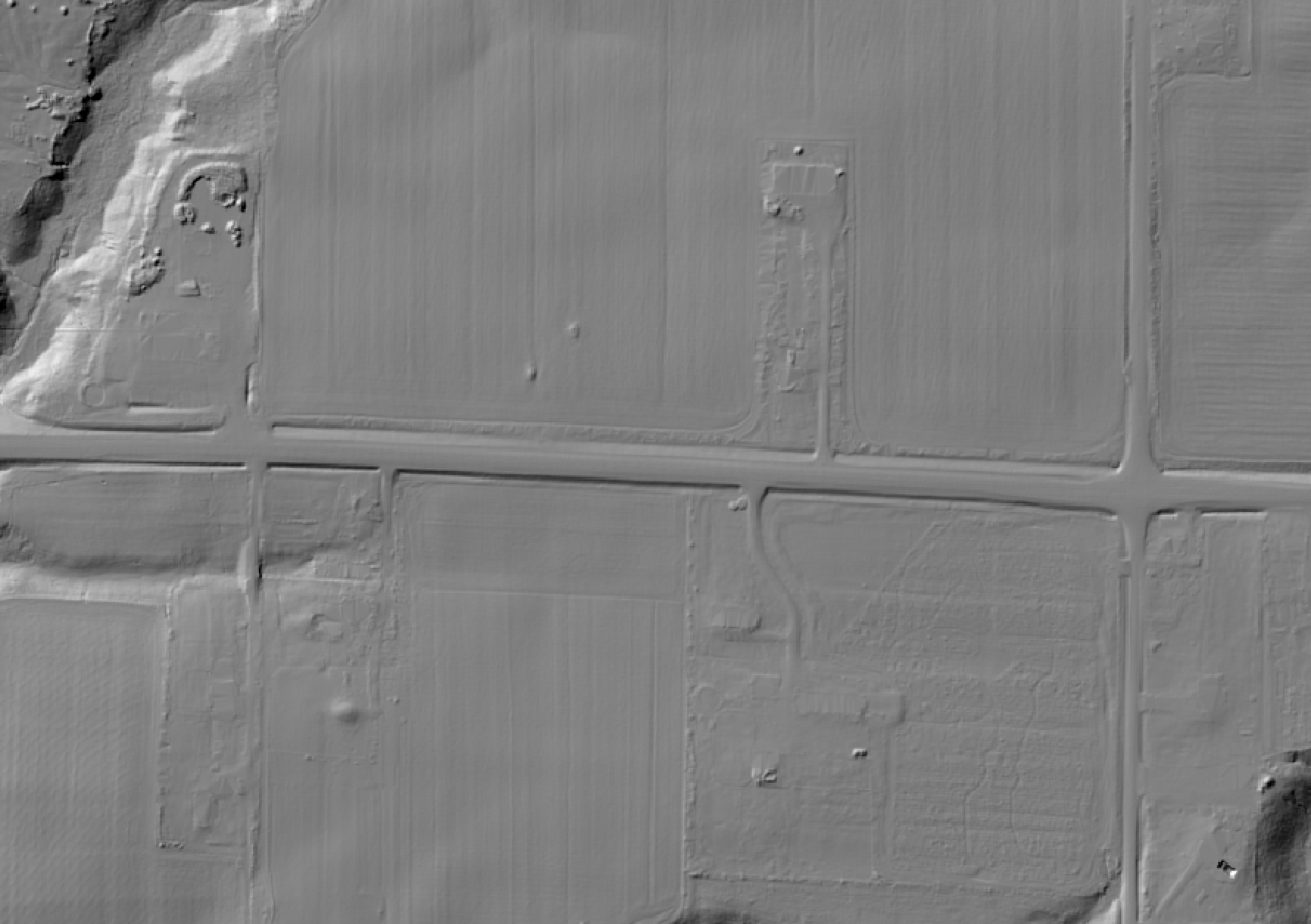Hillshade from embankment-removed DEM: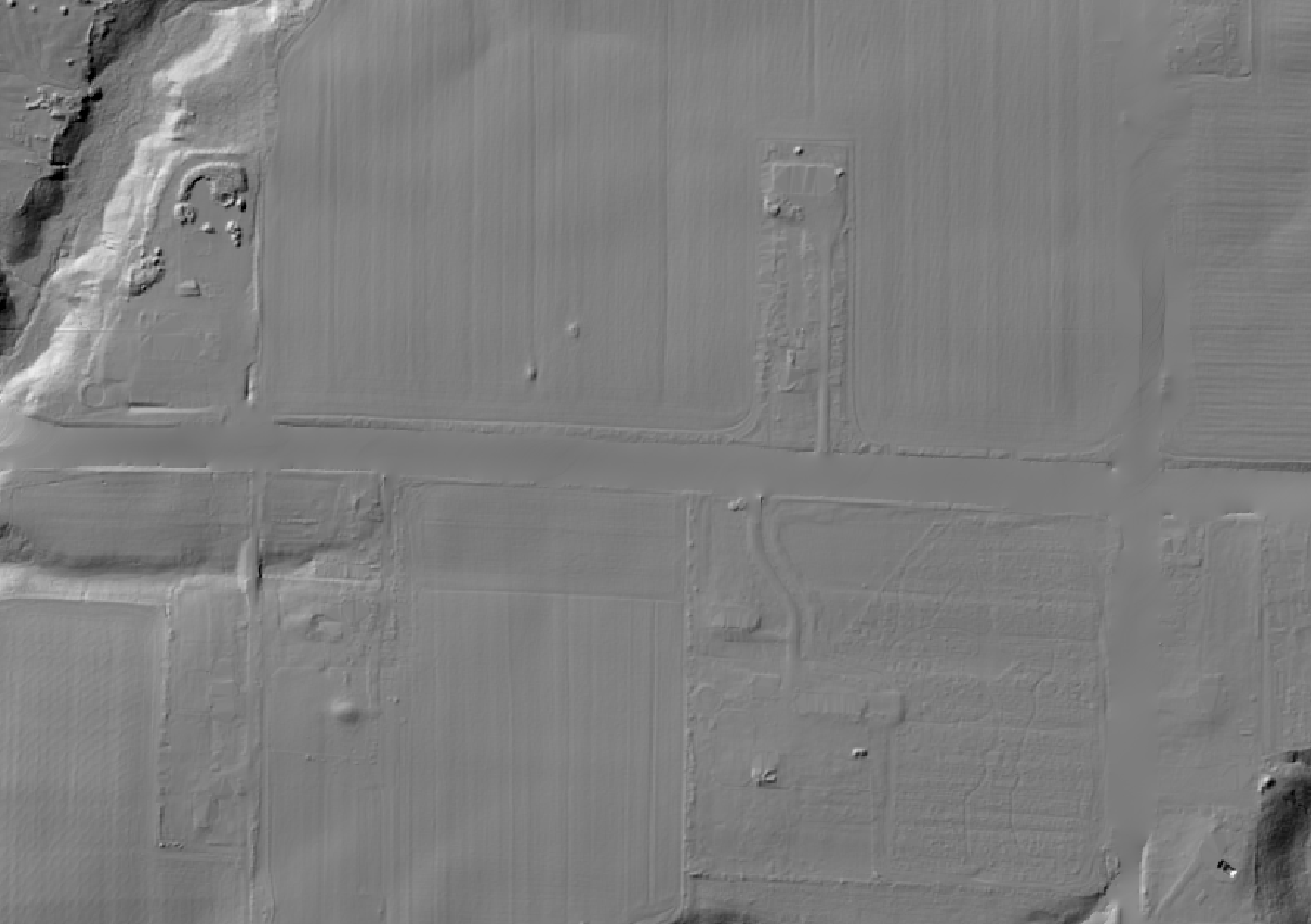References:

Van Nieuwenhuizen, N, Lindsay, JB, DeVries, B. 2021. Automated mapping of transportation embankments in fine-resolution LiDAR DEMs. Remote Sensing. 13(7), 1308; https://doi.org/10.3390/rs13071308

Parameters:

FlagDescription
-i, --demInput raster DEM file
-o, --outputOutput raster file
--search_distSearch distance used to reposition transportation vectors onto road embankments (in map units)
--typical_widthTypical embankment width; this is the maximum width of an embankment with roadside ditches (in map units)
--max_heightTypical embankment maximum height; this is the height a typical embankment with roadside ditches (in map units)
--max_widthMaximum embankment width, typically where embankments traverse steep-sided valleys (in map units)
--max_incrementMaximum upwards increment between neighbouring cells on an embankment (in elevation units)
--spillout_slopeSpillout slope (in degrees)
--remove_embankmentsOptional flag indicating whether to output a DEM with embankments removed (true) or an embankment raster map (false)

Python function:

``````wbt.embankment_mapping(
dem,
output,
search_dist=2.5,
typical_width=30.0,
max_height=2.0,
max_width=60.0,
max_increment=0.05,
spillout_slope=4.0,
remove_embankments=False,
callback=default_callback
)
``````

Command-line Interface:

``````>>./whitebox_tools -r=EmbankmentMapping -v ^
--wd="/path/to/data/" -i=DEM.tif -o=output.tif ^
--max_height=2.0 --max_width=60.0 --max_increment=0.05 ^
--spillout_slope=4.0 --remove_embankments=true
``````

Source code on GitHub

Author: Dr. John Lindsay and Nigel Van Nieuwenhuizen

Created: 21/09/2020

# FeaturePreservingSmoothing

This tool implements a highly modified form of the DEM de-noising algorithm described by Sun et al. (2007). It is very effective at removing surface roughness from digital elevation models (DEMs), without significantly altering breaks-in-slope. As such, this tool should be used for smoothing DEMs rather than either smoothing with low-pass filters (e.g. mean, median, Gaussian filters) or grid size coarsening by resampling. The algorithm works by 1) calculating the surface normal 3D vector of each grid cell in the DEM, 2) smoothing the normal vector field using a filtering scheme that applies more weight to neighbours with lower angular difference in surface normal vectors, and 3) uses the smoothed normal vector field to update the elevations in the input DEM.

Sun et al.'s (2007) original method was intended to work on input point clouds and fitted triangular irregular networks (TINs). The algorithm has been modified to work with input raster DEMs instead. In so doing, this algorithm calculates surface normal vectors from the planes fitted to 3 x 3 neighbourhoods surrounding each grid cell, rather than the triangular facet. The normal vector field smoothing and elevation updating procedures are also based on raster filtering operations. These modifications make this tool more efficient than Sun's original method, but will also result in a slightly different output than what would be achieved with Sun's method.

The user must specify the values of three key parameters, including the filter size (`--filter`), the normal difference threshold (`--norm_diff`), and the number of iterations (`--num_iter`). Lindsay et al. (2019) found that the degree of smoothing was less impacted by the filter size than it was either the normal difference threshold and the number of iterations. A filter size of 11, the default value, tends to work well in many cases. To increase the level of smoothing applied to the DEM, consider increasing the normal difference threshold, i.e. the angular difference in normal vectors between the center cell of a filter window and a neighbouring cell. This parameter determines which neighbouring values are included in a filtering operation and higher values will result in a greater number of neighbouring cells included, and therefore smooother surfaces. Similarly, increasing the number of iterations from the default value of 3 to upwards of 5-10 will result in significantly greater smoothing.

Before smoothing treatment: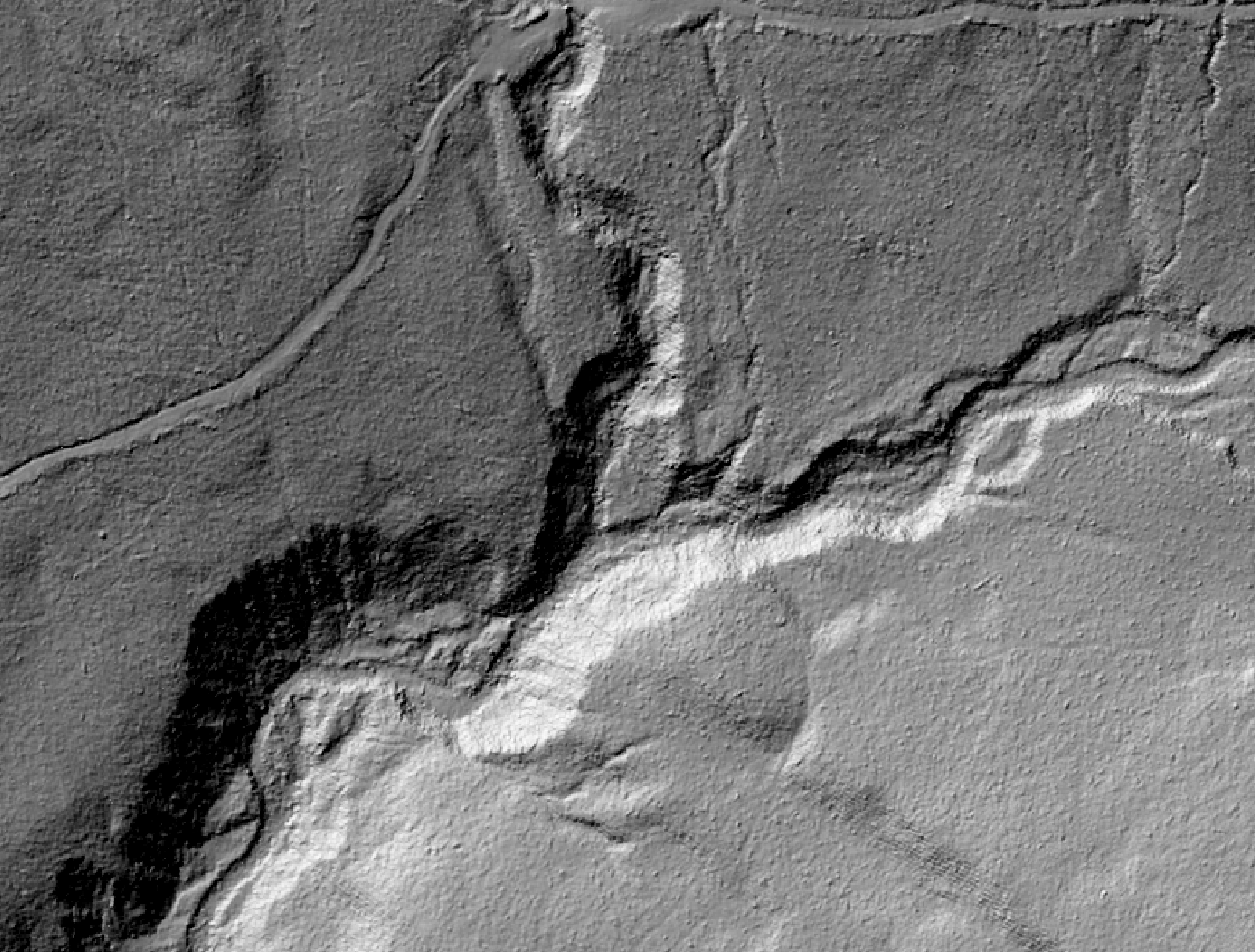After smoothing treatment with FPS: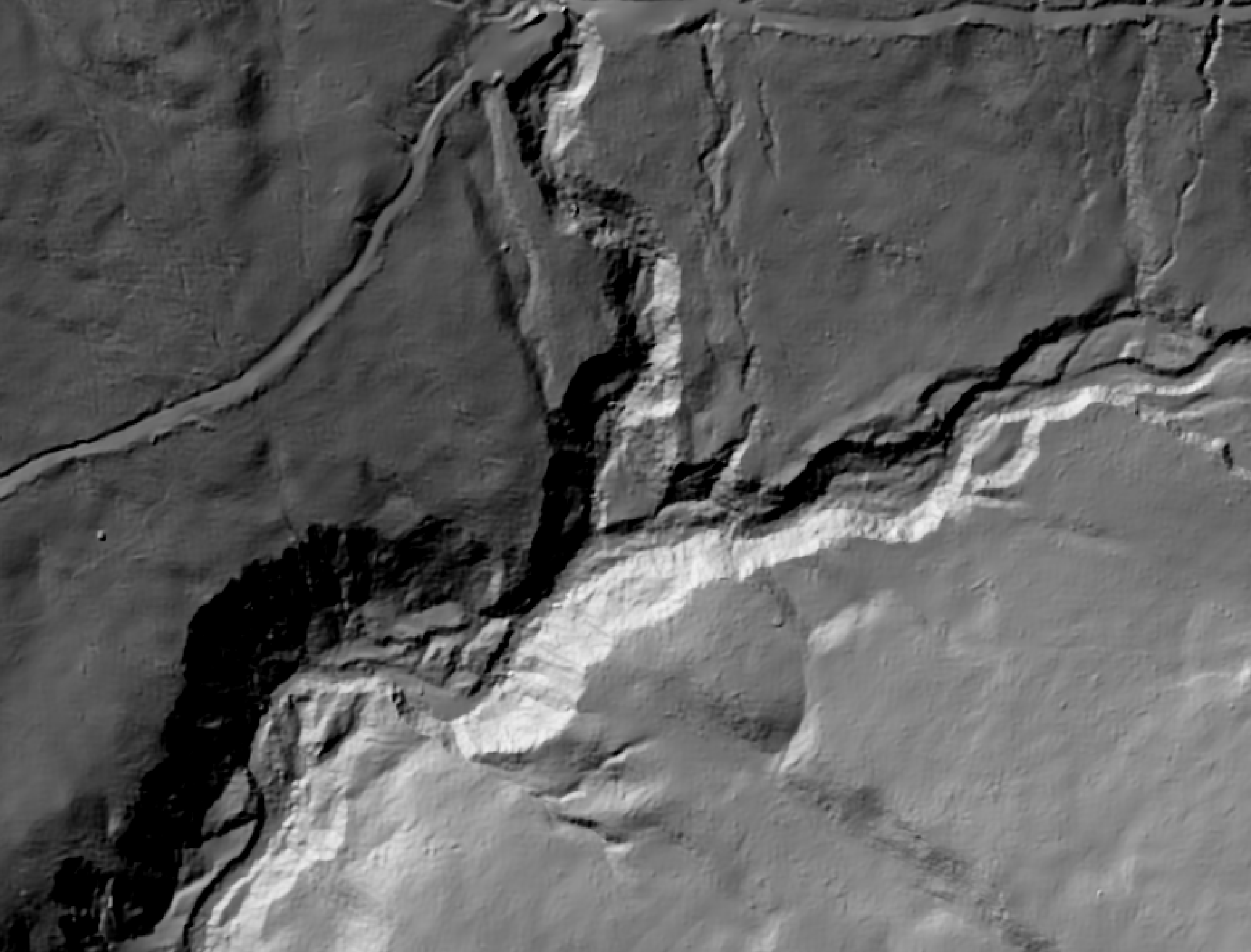Reference:

Lindsay JB, Francioni A, Cockburn JMH. 2019. LiDAR DEM smoothing and the preservation of drainage features. Remote Sensing, 11(16), 1926; DOI: 10.3390/rs11161926.

Sun, X., Rosin, P., Martin, R., & Langbein, F. (2007). Fast and effective feature-preserving mesh denoising. IEEE Transactions on Visualization & Computer Graphics, (5), 925-938.

Parameters:

FlagDescription
-i, --demInput raster DEM file
-o, --outputOutput raster file
--filterSize of the filter kernel
--norm_diffMaximum difference in normal vectors, in degrees
--num_iterNumber of iterations
--max_diffMaximum allowable absolute elevation change (optional)
--zfactorOptional multiplier for when the vertical and horizontal units are not the same

Python function:

``````wbt.feature_preserving_smoothing(
dem,
output,
filter=11,
norm_diff=15.0,
num_iter=3,
max_diff=0.5,
zfactor=None,
callback=default_callback
)
``````

Command-line Interface:

``````>>./whitebox_tools -r=FeaturePreservingSmoothing -v ^
--wd="/path/to/data/" --dem=DEM.tif -o=output.tif --filter=15 ^
--norm_diff=20.0 --num_iter=4
``````

Source code on GitHub

Author: Dr. John Lindsay

Created: 23/11/2017

# FetchAnalysis

This tool creates a new raster in which each grid cell is assigned the distance, in meters, to the nearest topographic obstacle in a specified direction. It is a modification of the algorithm described by Lapen and Martz (1993). Unlike the original algorithm, Fetch Analysis is capable of analyzing fetch in any direction from 0-360 degrees. The user must specify the name of an input digital elevation model (DEM) raster file, the output raster name, a hypothetical wind direction, and a value for the height increment parameter. The algorithm searches each grid cell in a path following the specified wind direction until the following condition is met:

Ztest >= Zcore + DI

where Zcore is the elevation of the grid cell at which fetch is being determined, Ztest is the elevation of the grid cell being tested as a topographic obstacle, D is the distance between the two grid cells in meters, and I is the height increment in m/m. Lapen and Martz (1993) suggest values for I in the range of 0.025 m/m to 0.1 m/m based on their study of snow re-distribution in low-relief agricultural landscapes of the Canadian Prairies. If the directional search does not identify an obstacle grid cell before the edge of the DEM is reached, the distance between the DEM edge and Zcore is entered. Edge distances are assigned negative values to differentiate between these artificially truncated fetch values and those for which a valid topographic obstacle was identified. Notice that linear interpolation is used to estimate the elevation of the surface where a ray (i.e. the search path) does not intersect the DEM grid precisely at one of its nodes.

Ray-tracing is a highly computationally intensive task and therefore this tool may take considerable time to operate for larger sized DEMs. This tool is parallelized to aid with computational efficiency. NoData valued grid cells in the input image will be assigned NoData values in the output image. Fetch Analysis images are best displayed using the blue-white-red bipolar palette to distinguish between the positive and negative values that are present in the output.

Reference:

Lapen, D. R., & Martz, L. W. (1993). The measurement of two simple topographic indices of wind sheltering-exposure from raster digital elevation models. Computers & Geosciences, 19(6), 769-779.

Parameters:

FlagDescription
-i, --demInput raster DEM file
-o, --outputOutput raster file
--azimuthWind azimuth in degrees in degrees
--hgt_incHeight increment value

Python function:

``````wbt.fetch_analysis(
dem,
output,
azimuth=0.0,
hgt_inc=0.05,
callback=default_callback
)
``````

Command-line Interface:

``````>>./whitebox_tools -r=FetchAnalysis -v --wd="/path/to/data/" ^
-i='input.tif' -o=output.tif --azimuth=315.0
``````

Source code on GitHub

Author: Dr. John Lindsay

Created: 07/07/2017

# FillMissingData

This tool can be used to fill in small gaps in a raster or digital elevation model (DEM). The gaps, or holes, must have recognized NoData values. If gaps do not currently have this characteristic, use the SetNodataValue tool and ensure that the data are stored using a raster format that supports NoData values. All valid, non-NoData values in the input raster will be assigned the same value in the output image.

The algorithm uses an inverse-distance weighted (IDW) scheme based on the valid values on the edge of NoData gaps to estimate gap values. The user must specify the filter size (`--filter`), which determines the size of gap that is filled, and the IDW weight (`--weight`).

The filter size, specified in grid cells, is used to determine how far the algorithm will search for valid, non-NoData values. Therefore, setting a larger filter size allows for the filling of larger gaps in the input raster.

The `--no_edges` flag can be used to exclude NoData values that are connected to the edges of the raster. It is usually the case that irregularly shaped DEMs have large regions of NoData values along the containing raster edges. This flag can be used to exclude these regions from the gap-filling operation, leaving only interior gaps for filling.

Parameters:

FlagDescription
-i, --inputInput raster file
-o, --outputOutput raster file
--filterFilter size (cells)
--weightIDW weight value
--no_edgesOptional flag indicating whether to exclude NoData cells in edge regions

Python function:

``````wbt.fill_missing_data(
i,
output,
filter=11,
weight=2.0,
no_edges=True,
callback=default_callback
)
``````

Command-line Interface:

``````>>./whitebox_tools -r=FillMissingData -v ^
--wd="/path/to/data/" -i=DEM.tif -o=output.tif --filter=25 ^
--weight=1.0 --no_edges
``````

Source code on GitHub

Author: Dr. John Lindsay

Created: 14/06/2017

# FindRidges

This tool can be used to identify ridge cells in a digital elevation model (DEM). Ridge cells are those that have lower neighbours either to the north and south or the east and west. Line thinning can optionally be used to create single-cell wide ridge networks by specifying the `--line_thin` parameter.

Parameters:

FlagDescription
-i, --demInput raster DEM file
-o, --outputOutput raster file
--line_thinOptional flag indicating whether post-processing line-thinning should be performed

Python function:

``````wbt.find_ridges(
dem,
output,
line_thin=True,
callback=default_callback
)
``````

Command-line Interface:

``````>>./whitebox_tools -r=FindRidges -v --wd="/path/to/data/" ^
--dem=pointer.tif -o=out.tif --line_thin
``````

Source code on GitHub

Author: Dr. John Lindsay

Created: 04/12/2017

This tool performs a hillshade operation (also called shaded relief) on an input digital elevation model (DEM). The user must specify the name of the input DEM and the output hillshade image name. Other parameters that must be specified include the illumination source azimuth (`--azimuth`), or sun direction (0-360 degrees), the illumination source altitude (`--altitude`; i.e. the elevation of the sun above the horizon, measured as an angle from 0 to 90 degrees) and the Z conversion factor (`--zfactor`). The Z conversion factor is only important when the vertical and horizontal units are not the same in the DEM. When this is the case, the algorithm will multiply each elevation in the DEM by the Z conversion factor. If the DEM is in the geographic coordinate system (latitude and longitude), the following equation is used:

zfactor = 1.0 / (111320.0 x cos(mid_lat))

where `mid_lat` is the latitude of the centre of the raster, in radians.

The hillshade value (HS) of a DEM grid cell is calculate as:

HS = tan(s) / [1 - tan(s)2]0.5 x [sin(Alt) / tan(s) - cos(Alt) x sin(Az - a)]

where s and a are the local slope gradient and aspect (orientation) respectively and Alt and Az are the illumination source altitude and azimuth respectively. Slope and aspect are calculated using Horn's (1981) 3rd-order finate difference method.

Reference:

Gallant, J. C., and J. P. Wilson, 2000, Primary topographic attributes, in Terrain Analysis: Principles and Applications, edited by J. P. Wilson and J. C. Gallant pp. 51-86, John Wiley, Hoboken, N.J.

Parameters:

FlagDescription
-i, --demInput raster DEM file
-o, --outputOutput raster file
--azimuthIllumination source azimuth in degrees
--altitudeIllumination source altitude in degrees
--zfactorOptional multiplier for when the vertical and horizontal units are not the same

Python function:

``````wbt.hillshade(
dem,
output,
azimuth=315.0,
altitude=30.0,
zfactor=None,
callback=default_callback
)
``````

Command-line Interface:

``````>>./whitebox_tools -r=Hillshade -v --wd="/path/to/data/" ^
-i=DEM.tif -o=output.tif --azimuth=315.0 --altitude=30.0
``````

Source code on GitHub

Author: Dr. John Lindsay

Created: 22/06/2017

# HorizonAngle

This tool calculates the horizon angle (Sx), i.e. the maximum slope along a specified azimuth (0-360 degrees) for each grid cell in an input digital elevation model (DEM). Horizon angle is sometime referred to as the maximum upwind slope in wind exposure/sheltering studies. Positive values can be considered sheltered with respect to the azimuth and negative values are exposed. Thus, Sx is a measure of exposure to a wind from a specific direction. The algorithm works by tracing a ray from each grid cell in the direction of interest and evaluating the slope for each location in which the DEM grid is intersected by the ray. Linear interpolation is used to estimate the elevation of the surface where a ray does not intersect the DEM grid precisely at one of its nodes.

The user is able to constrain the maximum search distance (`--max_dist`) for the ray tracing by entering a valid maximum search distance value (in the same units as the X-Y coordinates of the input raster DEM). If the maximum search distance is left blank, each ray will be traced to the edge of the DEM, which will add to the computational time.

Maximum upwind slope should not be calculated for very extensive areas over which the Earth's curvature must be taken into account. Also, this index does not take into account the deflection of wind by topography. However, averaging the horizon angle over a window of directions can yield a more robust measure of exposure, compensating for the deflection of wind from its regional average by the topography. For example, if you are interested in measuring the exposure of a landscape to a northerly wind, you could perform the following calculation:

Sx(N) = [Sx(345)+Sx(350)+Sx(355)+Sx(0)+Sx(5)+Sx(10)+Sx(15)] / 7.0

Ray-tracing is a highly computationally intensive task and therefore this tool may take considerable time to operate for larger sized DEMs. Maximum upwind slope is best displayed using a Grey scale palette that is inverted.

Horizon angle is best visualized using a white-to-black palette and rescaled from approximately -10 to 70 (see below for an example of horizon angle calculated at a 150-degree azimuth).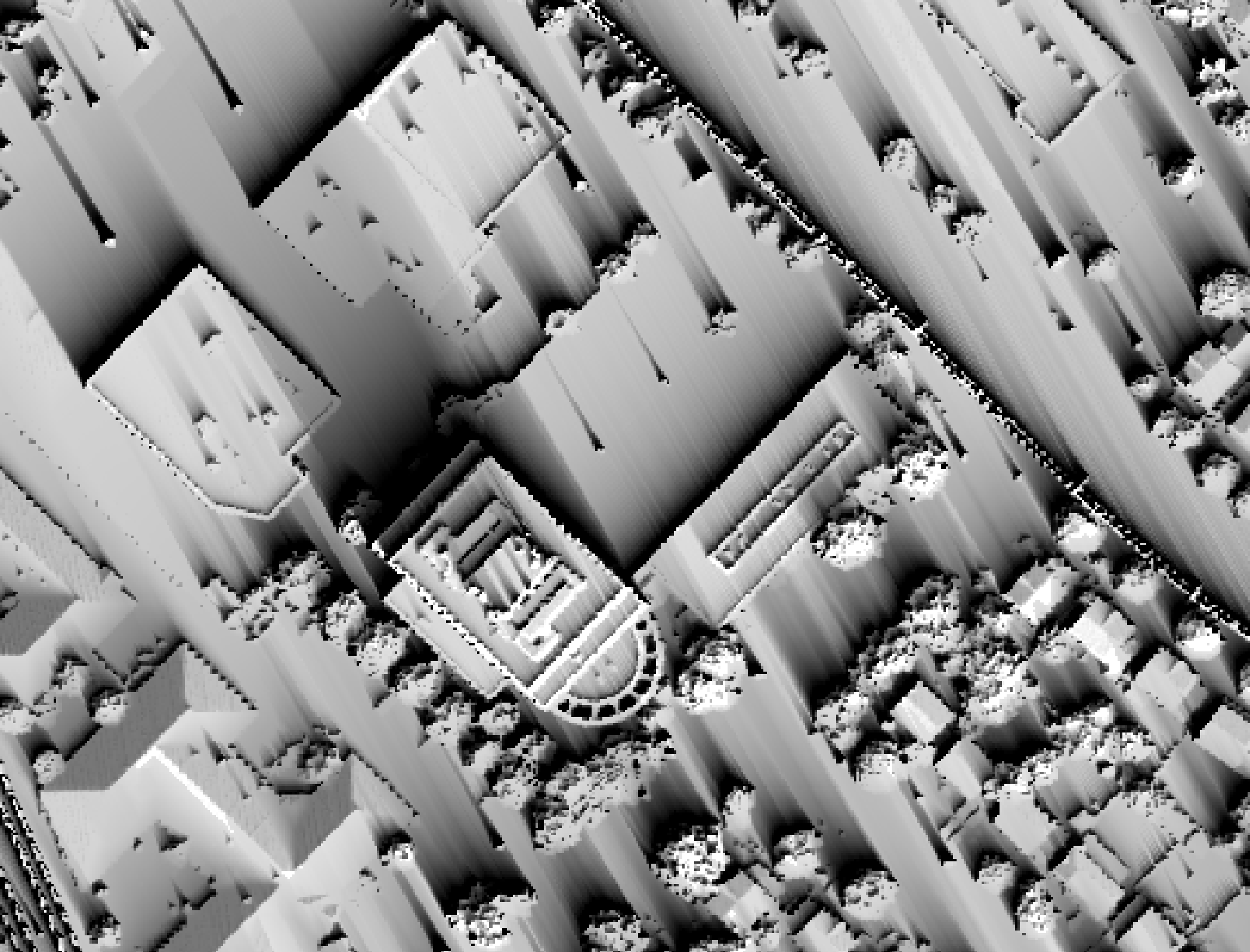Parameters:

FlagDescription
-i, --demInput raster DEM file
-o, --outputOutput raster file
--azimuthAzimuth, in degrees
--max_distOptional maximum search distance (unspecified if none; in xy units). Minimum value is 5 x cell size

Python function:

``````wbt.horizon_angle(
dem,
output,
azimuth=0.0,
max_dist=100.0,
callback=default_callback
)
``````

Command-line Interface:

``````>>./whitebox_tools -r=HorizonAngle -v --wd="/path/to/data/" ^
-i='input.tif' -o=output.tif --azimuth=315.0
``````

Source code on GitHub

Author: Dr. John Lindsay

Created: 07/07/2017

# HypsometricAnalysis

This tool can be used to derive the hypsometric curve, or area-altitude curve, of one or more input digital elevation models (DEMs) (`--inputs`). A hypsometric curve is a histogram or cumulative distribution function of elevations in a geographical area.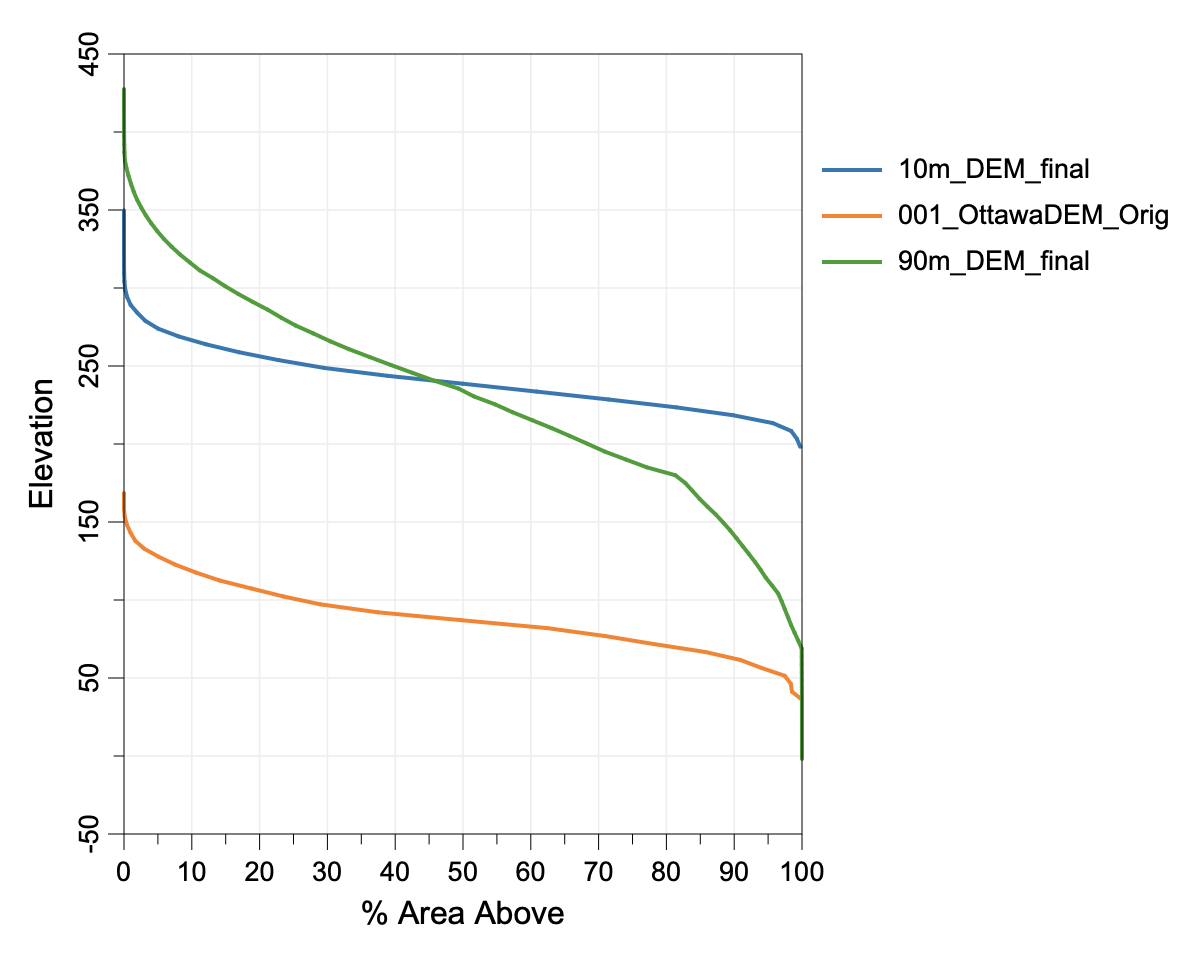Parameters:

FlagDescription
-i, --inputsInput DEM files
--watershedInput watershed files (optional)
-o, --outputOutput HTML file (default name will be based on input file if unspecified)

Python function:

``````wbt.hypsometric_analysis(
inputs,
output,
watershed=None,
callback=default_callback
)
``````

Command-line Interface:

``````>>./whitebox_tools -r=HypsometricAnalysis -v ^
--wd="/path/to/data/" -i="DEM1.tif;DEM2.tif" ^
--watershed="ws1.tif;ws2.tif" -o=outfile.html
``````

Source code on GitHub

Author: Dr. John Lindsay

Created: 30/01/2018

This tool creates a hypsometrically tinted shaded relief (Swiss hillshading) image from an input digital elevation model (DEM). The tool combines a colourized version of the DEM with varying illumination provided by a hillshade image, to produce a composite relief model that can be used to visual topography for more effective interpretation of landscapes. The output (`--output`) of the tool is a 24-bit red-green-blue (RGB) colour image.

The user must specify the name of the input DEM and the output image name. Other parameters that must be specified include the illumination source azimuth (`--azimuth`), or sun direction (0-360 degrees), the illumination source altitude (`--altitude`; i.e. the elevation of the sun above the horizon, measured as an angle from 0 to 90 degrees), the hillshade weight (`--hs_weight`; 0-1), image brightness (`--brightness`; 0-1), and atmospheric effects (`--atmospheric`; 0-1). The hillshade weight can be used to increase or subdue the relative prevalence of the hillshading effect in the output image. The image brightness parameter is used to create an overall brighter or darker version of the terrain rendering; note however, that very high values may over-saturate the well-illuminated portions of the terrain. The atmospheric effects parameter can be used to introduce a haze or atmosphere effect to the output image. It is intended to reproduce the effect of viewing mountain valley bottoms through a thicker and more dense atmosphere. Values greater than zero will introduce a slightly blue tint, particularly at lower altitudes, blur the hillshade edges slightly, and create a random haze-like speckle in lower areas. The user must also specify the Z conversion factor (`--zfactor`). The Z conversion factor is only important when the vertical and horizontal units are not the same in the DEM. When this is the case, the algorithm will multiply each elevation in the DEM by the Z conversion factor. If the DEM is in the geographic coordinate system (latitude and longitude), the following equation is used:

zfactor = 1.0 / (111320.0 x cos(mid_lat))

where `mid_lat` is the latitude of the centre of the raster, in radians.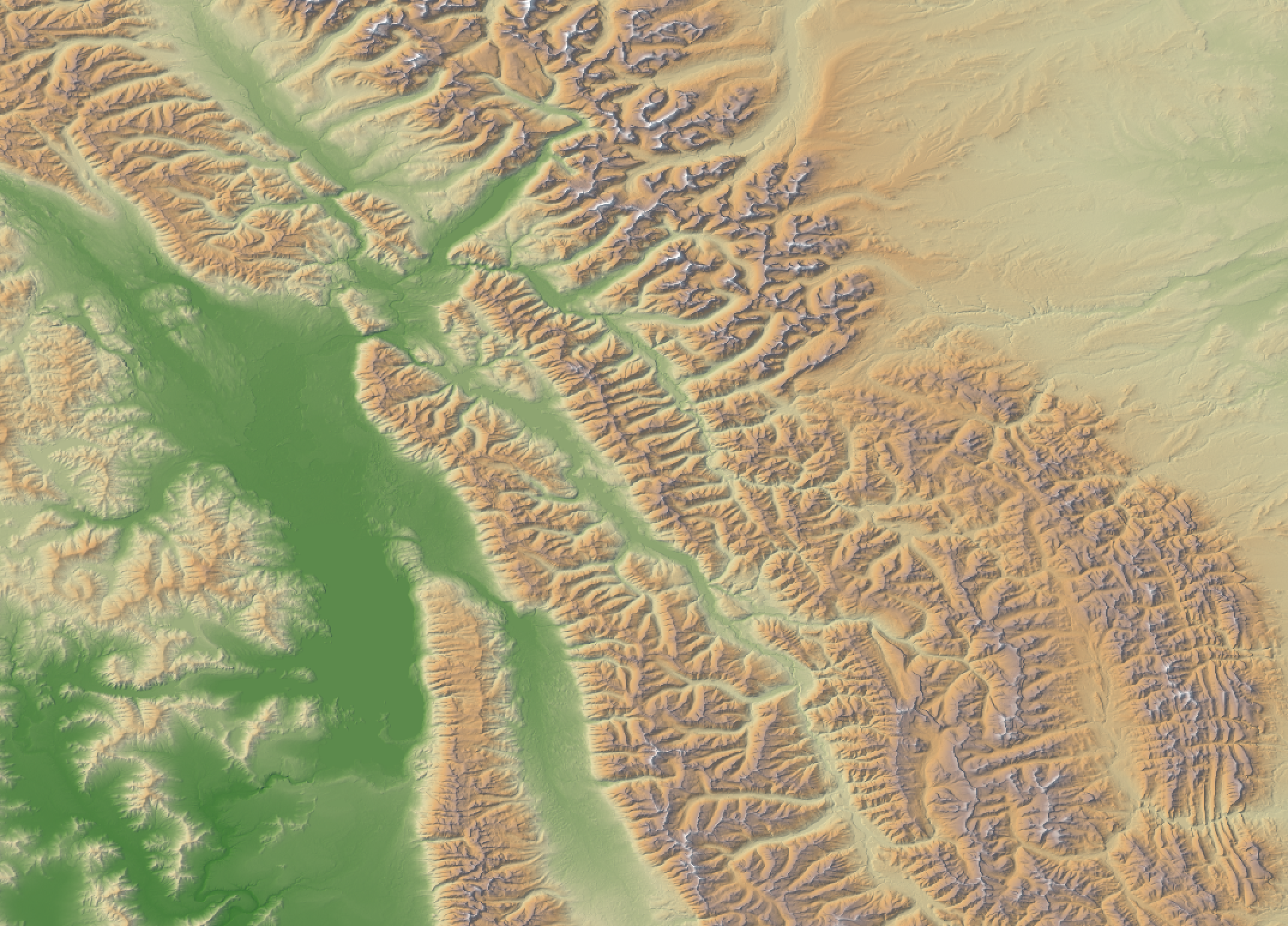Parameters:

FlagDescription
-i, --demInput raster DEM file
-o, --outputOutput raster file
--altitudeIllumination source altitude in degrees
--hs_weightWeight given to hillshade relative to relief (0.0-1.0)
--brightnessBrightness factor (0.0-1.0)
--atmosphericAtmospheric effects weight (0.0-1.0)
--paletteOptions include 'atlas', 'high_relief', 'arid', 'soft', 'muted', 'purple', 'viridi', 'gn_yl', 'pi_y_g', 'bl_yl_rd', and 'deep'
--reverseOptional flag indicating whether to use reverse the palette
--zfactorOptional multiplier for when the vertical and horizontal units are not the same
--full_modeOptional flag indicating whether to use full 360-degrees of illumination sources

Python function:

``````wbt.hypsometrically_tinted_hillshade(
dem,
output,
altitude=45.0,
hs_weight=0.5,
brightness=0.5,
atmospheric=0.0,
palette="atlas",
reverse=False,
zfactor=None,
full_mode=False,
callback=default_callback
)
``````

Command-line Interface:

``````>>./whitebox_tools -r=HypsometricallyTintedHillshade -v ^
--wd="/path/to/data/" -i=DEM.tif -o=output.tif --altitude=45.0 ^
--hs_weight=0.3 --brightness=0.6 --atmospheric=0.2 ^
--palette=arid --full_mode
``````

Source code on GitHub

Author: Dr. John Lindsay

Created: 09/07/2020

# MapOffTerrainObjects

This tool can be used to map off-terrain objects in a digital surface model (DSM) based on cell-to-cell differences in elevations and local slopes. The algorithm works by using a region-growing operation to connect neighbouring grid cells outwards from seed cells. Two neighbouring cells are considered connected if the slope between the two cells is less than the user-specified maximum slope value (`--max_slope`). Mapped segments that are less than the minimum feature size (`--min_size`), in grid cells, are assigned a common background value. Note that this method of mapping off-terrain objects, and thereby separating ground cells from non-ground objects in DSMs, works best with fine-resolution DSMs that have been interpolated using a non-smoothing method, such as triangulation (TINing) or nearest-neighbour interpolation.

Parameters:

FlagDescription
-i, --demInput raster DEM file
-o, --outputOutput raster file
--max_slopeMaximum inter-cell absolute slope
--min_sizeMinimum feature size, in grid cells

Python function:

``````wbt.map_off_terrain_objects(
dem,
output,
max_slope=40.0,
min_size=1,
callback=default_callback
)
``````

Command-line Interface:

``````>>./whitebox_tools -r=MapOffTerrainObjects -v ^
--wd="/path/to/data/" --dem=DEM.tif -o=output.tif ^
--max_diff=1.0
``````

Source code on GitHub

Author: Dr. John Lindsay

Created: 27/07/2020

# MaxAnisotropyDev

Calculates the maximum anisotropy (directionality) in elevation deviation over a range of spatial scales.

Parameters:

FlagDescription
-i, --demInput raster DEM file
--out_magOutput raster DEVmax magnitude file
--out_scaleOutput raster DEVmax scale file
--min_scaleMinimum search neighbourhood radius in grid cells
--max_scaleMaximum search neighbourhood radius in grid cells
--stepStep size as any positive non-zero integer

Python function:

``````wbt.max_anisotropy_dev(
dem,
out_mag,
out_scale,
max_scale,
min_scale=3,
step=2,
callback=default_callback
)
``````

Command-line Interface:

``````>>./whitebox_tools -r=MaxAnisotropyDev -v ^
--wd="/path/to/data/" --dem=DEM.tif --out_mag=DEVmax_mag.tif ^
--out_scale=DEVmax_scale.tif --min_scale=1 --max_scale=1000 ^
--step=5
``````

Source code on GitHub

Author: Dan Newman and John Lindsay

Created: January 26, 2018

# MaxAnisotropyDevSignature

Calculates the anisotropy in deviation from mean for points over a range of spatial scales.

Parameters:

FlagDescription
-i, --demInput raster DEM file
--pointsInput vector points file
-o, --outputOutput HTML file
--min_scaleMinimum search neighbourhood radius in grid cells
--max_scaleMaximum search neighbourhood radius in grid cells
--stepStep size as any positive non-zero integer

Python function:

``````wbt.max_anisotropy_dev_signature(
dem,
points,
output,
max_scale,
min_scale=1,
step=1,
callback=default_callback
)
``````

Command-line Interface:

``````>>./whitebox_tools -r=MaxAnisotropyDevSignature -v ^
--wd="/path/to/data/" --dem=DEM.tif --points=sites.shp ^
--output=roughness.html --min_scale=1 --max_scale=1000 ^
--step=5
``````

Source code on GitHub

Author: Dan Newman and John Lindsay

Created: 27/03/2018

# MaxBranchLength

Maximum branch length (`Bmax`) is the longest branch length between a grid cell's flowpath and the flowpaths initiated at each of its neighbours. It can be conceptualized as the downslope distance that a volume of water that is split into two portions by a drainage divide would travel before reuniting.

If the two flowpaths of neighbouring grid cells do not intersect, `Bmax` is simply the flowpath length from the starting cell to its terminus at the edge of the grid or a cell with undefined flow direction (i.e. a pit cell either in a topographic depression or at the edge of a major body of water).

The pattern of `Bmax` derived from a DEM should be familiar to anyone who has interpreted upslope contributing area images. In fact, `Bmax` can be thought of as the complement of upslope contributing area. Whereas contributing area is greatest along valley bottoms and lowest at drainage divides, `Bmax` is greatest at divides and lowest along channels. The two topographic attributes are also distinguished by their units of measurements; `Bmax` is a length rather than an area. The presence of a major drainage divide between neighbouring grid cells is apparent in a `Bmax` image as a linear feature, often two grid cells wide, of relatively high values. This property makes `Bmax` a useful land surface parameter for mapping ridges and divides.

`Bmax` is useful in the study of landscape structure, particularly with respect to drainage patterns. The index gives the relative significance of a specific location along a divide, with respect to the dispersion of materials across the landscape, in much the same way that stream ordering can be used to assess stream size.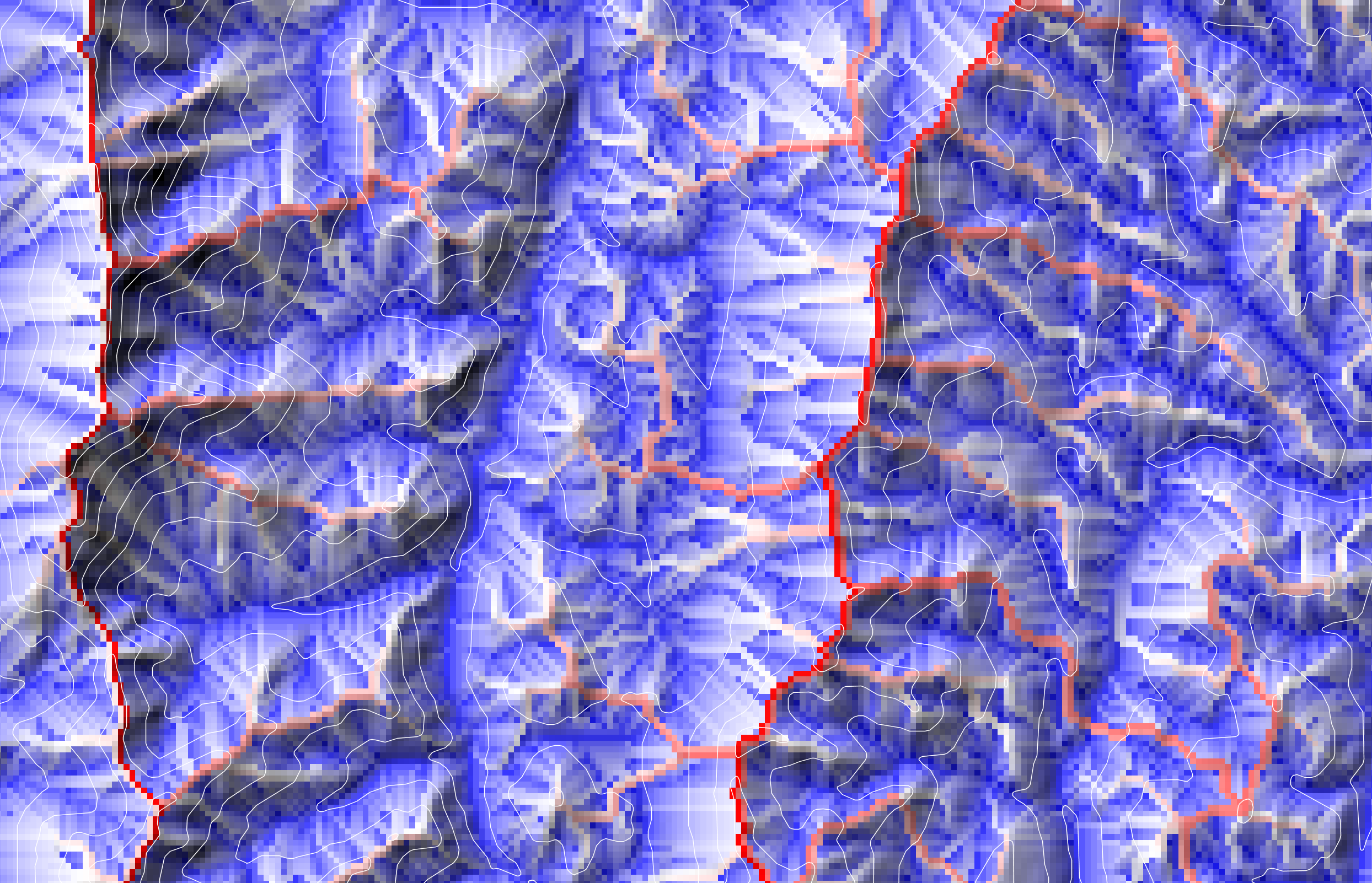Reference:

Lindsay JB, Seibert J. 2013. Measuring the significance of a divide to local drainage patterns. International Journal of Geographical Information Science, 27: 1453-1468. DOI: 10.1080/13658816.2012.705289

Parameters:

FlagDescription
-i, --demInput raster DEM file
-o, --outputOutput raster file
--logOptional flag to request the output be log-transformed

Python function:

``````wbt.max_branch_length(
dem,
output,
log=False,
callback=default_callback
)
``````

Command-line Interface:

``````>>./whitebox_tools -r=MaxBranchLength -v ^
--wd="/path/to/data/" --dem=DEM.tif -o=output.tif
``````

Source code on GitHub

Author: Dr. John Lindsay

Created: 09/07/2017

# MaxDifferenceFromMean

Calculates the maximum difference from mean elevation over a range of spatial scales.

Parameters:

FlagDescription
-i, --demInput raster DEM file
--out_magOutput raster DIFFmax magnitude file
--out_scaleOutput raster DIFFmax scale file
--min_scaleMinimum search neighbourhood radius in grid cells
--max_scaleMaximum search neighbourhood radius in grid cells
--stepStep size as any positive non-zero integer

Python function:

``````wbt.max_difference_from_mean(
dem,
out_mag,
out_scale,
min_scale,
max_scale,
step=1,
callback=default_callback
)
``````

Command-line Interface:

``````>>./whitebox_tools -r=MaxDifferenceFromMean -v ^
--wd="/path/to/data/" --dem=DEM.tif --out_mag=DEVmax_mag.tif ^
--out_scale=DEVmax_scale.tif --min_scale=1 --max_scale=1000 ^
--step=5
``````

Source code on GitHub

Author: Dr. John Lindsay

Created: 28/08/2018

# MaxDownslopeElevChange

This tool calculates the maximum elevation drop between each grid cell and its neighbouring cells within a digital elevation model (DEM). The user must specify the name of the input DEM (`--dem`) and the output (`--output`) raster name.

Parameters:

FlagDescription
-i, --demInput raster DEM file
-o, --outputOutput raster file

Python function:

``````wbt.max_downslope_elev_change(
dem,
output,
callback=default_callback
)
``````

Command-line Interface:

``````>>./whitebox_tools -r=MaxDownslopeElevChange -v ^
--wd="/path/to/data/" --dem=DEM.tif -o=out.tif
``````

Source code on GitHub

Author: Dr. John Lindsay

Created: 11/07/2017

# MaxElevDevSignature

Calculates the maximum elevation deviation over a range of spatial scales and for a set of points.

Parameters:

FlagDescription
-i, --demInput raster DEM file
--pointsInput vector points file
-o, --outputOutput HTML file
--min_scaleMinimum search neighbourhood radius in grid cells
--max_scaleMaximum search neighbourhood radius in grid cells
--stepStep size as any positive non-zero integer

Python function:

``````wbt.max_elev_dev_signature(
dem,
points,
output,
min_scale,
max_scale,
step=10,
callback=default_callback
)
``````

Command-line Interface:

``````>>./whitebox_tools -r=MaxElevDevSignature -v ^
--wd="/path/to/data/" --dem=DEM.tif --points=sites.tif ^
--output=topo_position.html --min_scale=1 --max_scale=1000 ^
--step=5
``````

Source code on GitHub

Author: Dr. John Lindsay

Created: March 1, 2018

# MaxElevationDeviation

This tool can be used to calculate the maximum deviation from mean elevation, DEVmax (Lindsay et al. 2015) for each grid cell in a digital elevation model (DEM) across a range specified spatial scales. DEV is an elevation residual index and is essentially equivalent to a local elevation z-score. This attribute measures the relative topographic position as a fraction of local relief, and so is normalized to the local surface roughness. The multi-scaled calculation of DEVmax utilizes an integral image approach (Crow, 1984) to ensure highly efficient filtering that is invariant with filter size, which is the algorithm characteristic that allows for this densely sampled multi-scale analysis. In this way, MaxElevationDeviation allows users to estimate the locally optimal scale with which to estimate DEV on a pixel-by-pixel basis. This multi-scaled version of local topographic position can reveal significant terrain characteristics and can aid with soil, vegetation, landform, and other mapping applications that depend on geomorphometric characterization.

The user must specify the name (`--dem`) of the input digital elevation model (DEM). The range of scales that are evaluated in calculating DEVmax are determined by the user-specified `--min_scale`, `--max_scale`, and `--step` parameters. All filter radii between the minimum and maximum scales, increasing by `step`, will be evaluated. The scale parameters are in units of grid cells and specify kernel size "radii" (r), such that:

d = 2r + 1

That is, a radii of 1, 2, 3... yields a square filters of dimension (d) 3 x 3, 5 x 5, 7 x 7...

DEV is estimated at each tested filter size and every grid cell is assigned the maximum DEV value across the evaluated scales.

The user must specify the file names of two output rasters, including the magnitude (DEVmax) and a second raster the assigns each pixel the scale at which DEVmax is encountered (DEVscale). The DEVscale raster can be very useful for revealing multi-scale landscape structure.

Reference:

Lindsay J, Cockburn J, Russell H. 2015. An integral image approach to performing multi-scale topographic position analysis. Geomorphology, 245: 51-61.

Parameters:

FlagDescription
-i, --demInput raster DEM file
--out_magOutput raster DEVmax magnitude file
--out_scaleOutput raster DEVmax scale file
--min_scaleMinimum search neighbourhood radius in grid cells
--max_scaleMaximum search neighbourhood radius in grid cells
--stepStep size as any positive non-zero integer

Python function:

``````wbt.max_elevation_deviation(
dem,
out_mag,
out_scale,
min_scale,
max_scale,
step=1,
callback=default_callback
)
``````

Command-line Interface:

``````>>./whitebox_tools -r=MaxElevationDeviation -v ^
--wd="/path/to/data/" --dem=DEM.tif --out_mag=DEVmax_mag.tif ^
--out_scale=DEVmax_scale.tif --min_scale=1 --max_scale=1000 ^
--step=5
``````

Source code on GitHub

Author: Dr. John Lindsay

Created: July 20, 2017

# MinDownslopeElevChange

This tool calculates the minimum elevation drop between each grid cell and its neighbouring cells within a digital elevation model (DEM). The user must specify the name of the input DEM (`--dem`) and the output (`--output`) raster name.

Parameters:

FlagDescription
-i, --demInput raster DEM file
-o, --outputOutput raster file

Python function:

``````wbt.min_downslope_elev_change(
dem,
output,
callback=default_callback
)
``````

Command-line Interface:

``````>>./whitebox_tools -r=MinDownslopeElevChange -v ^
--wd="/path/to/data/" --dem=DEM.tif -o=out.tif
``````

Source code on GitHub

Author: Dr. John Lindsay

Created: 11/07/2017

This tool performs a hillshade operation (also called shaded relief) on an input digital elevation model (DEM) with multiple sources of illumination. The user must specify the name of the input DEM (`--dem`) and the output hillshade image name (`--output`). Other parameters that must be specified include the altitude of the illumination sources (`--altitude`; i.e. the elevation of the sun above the horizon, measured as an angle from 0 to 90 degrees) and the Z conversion factor (`--zfactor`). The Z conversion factor is only important when the vertical and horizontal units are not the same in the DEM. When this is the case, the algorithm will multiply each elevation in the DEM by the Z conversion factor. If the DEM is in the geographic coordinate system (latitude and longitude), the following equation is used:

zfactor = 1.0 / (111320.0 x cos(mid_lat))

where `mid_lat` is the latitude of the centre of the raster, in radians. The Z conversion factor can also be used used to apply a vertical exageration to further emphasize landforms within the hillshade output.

The hillshade value (HS) of a DEM grid cell is calculate as:

HS = tan(s) / [1 - tan(s)2]0.5 x [sin(Alt) / tan(s) - cos(Alt) x sin(Az - a)]

where s and a are the local slope gradient and aspect (orientation) respectively and Alt and Az are the illumination source altitude and azimuth respectively. Slope and aspect are calculated using Horn's (1981) 3rd-order finate difference method.

Lastly, the user must specify whether or not to use full 360-degrees of illumination sources (`--full_mode`). When this flag is not specified, the tool will perform a weighted summation of the hillshade images from four illumination azimuth positions at 225, 270, 315, and 360 (0) degrees, given weights of 0.1, 0.4, 0.4, and 0.1 respectively. When run in the full 360-degree mode, eight illumination source azimuths are used to calculate the output at 0, 45, 90, 135, 180, 225, 270, and 315 degrees, with weights of 0.15, 0.125, 0.1, 0.05, 0.1, 0.125, 0.15, and 0.2 respectively.

Classic hillshade (Azimuth=315, Altitude=45.0)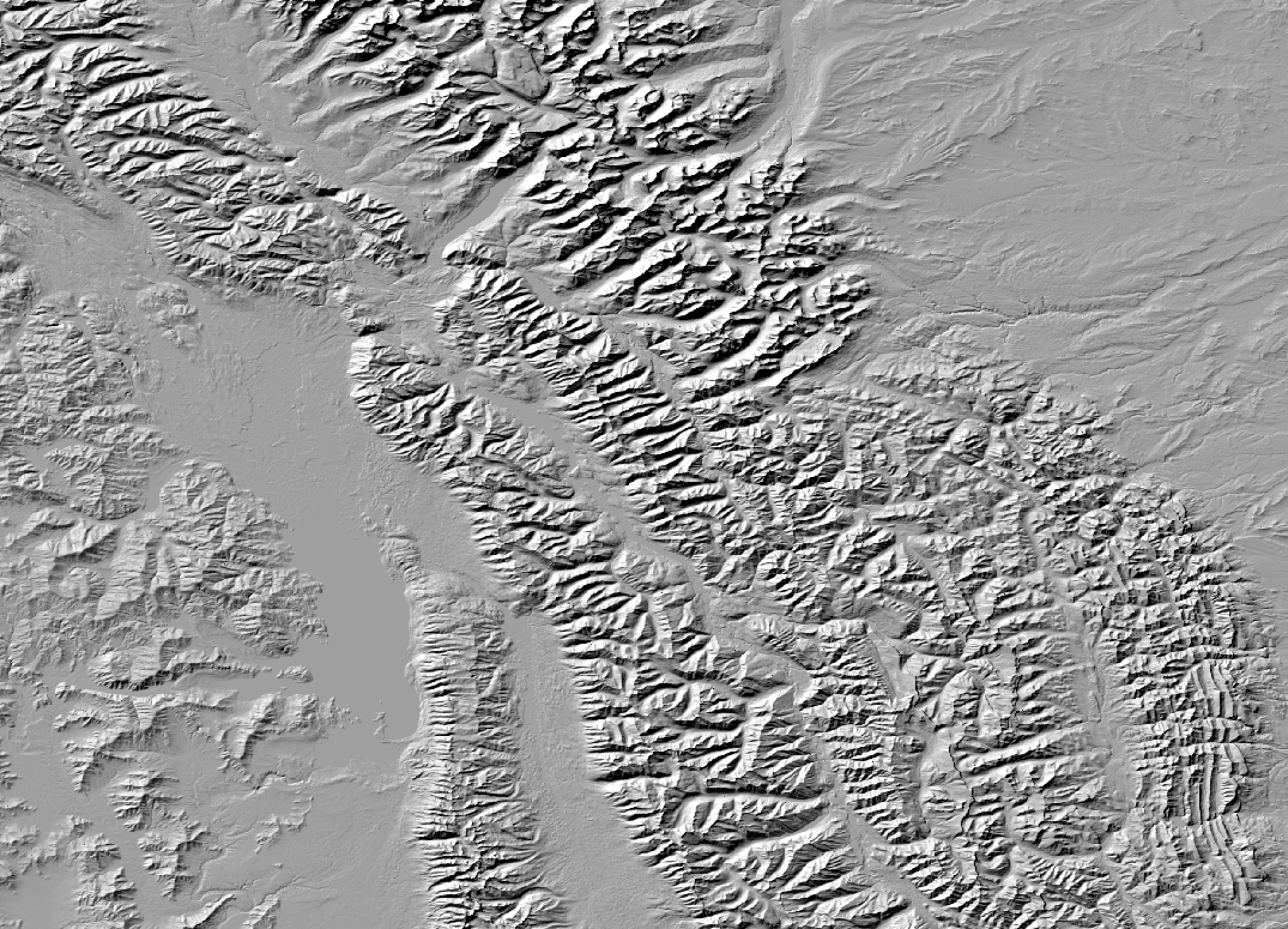Multi-directional hillshade (Altitude=45.0, Four-direction mode)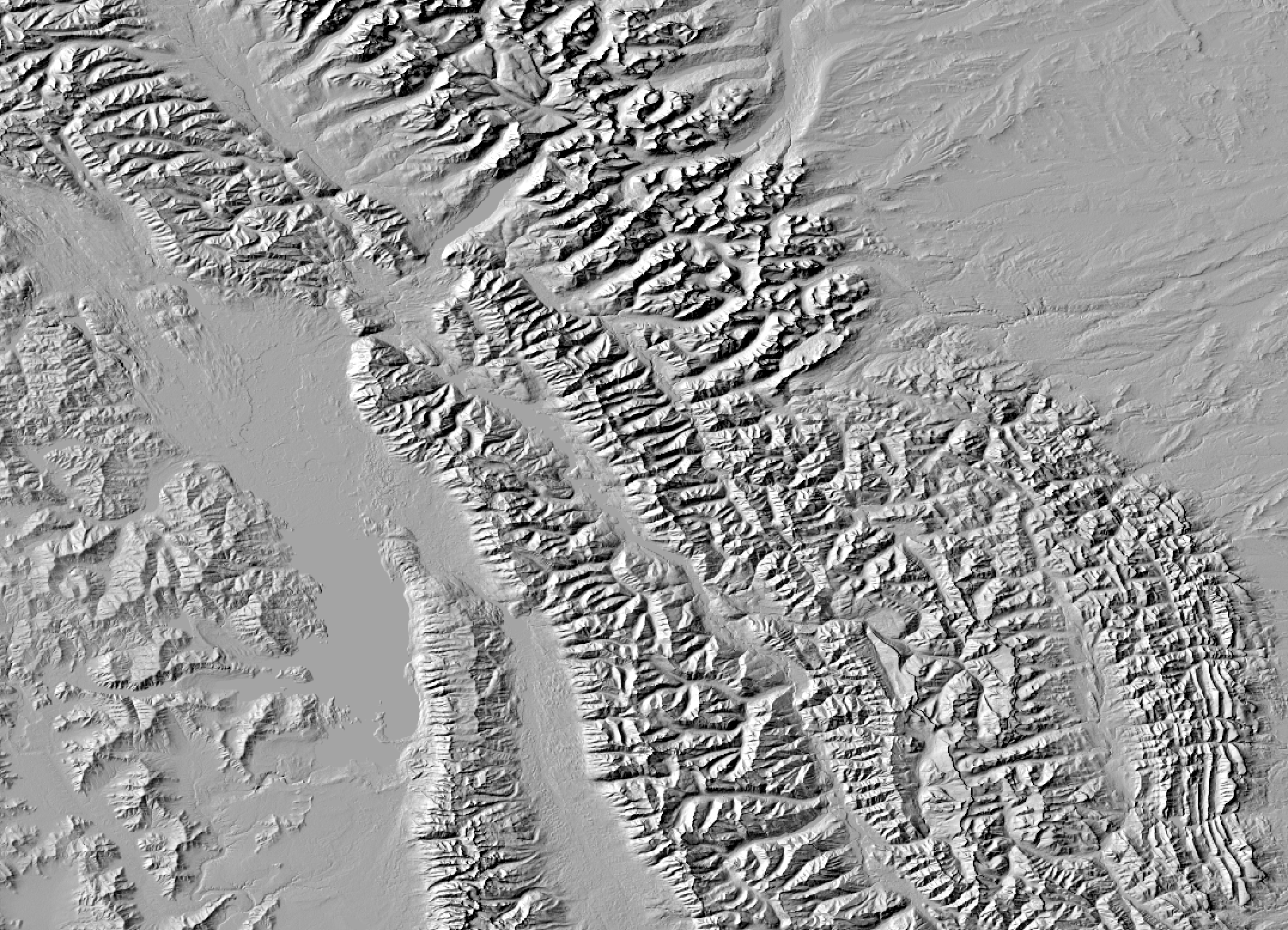Multi-directional hillshade (Altitude=45.0, 360-degree mode)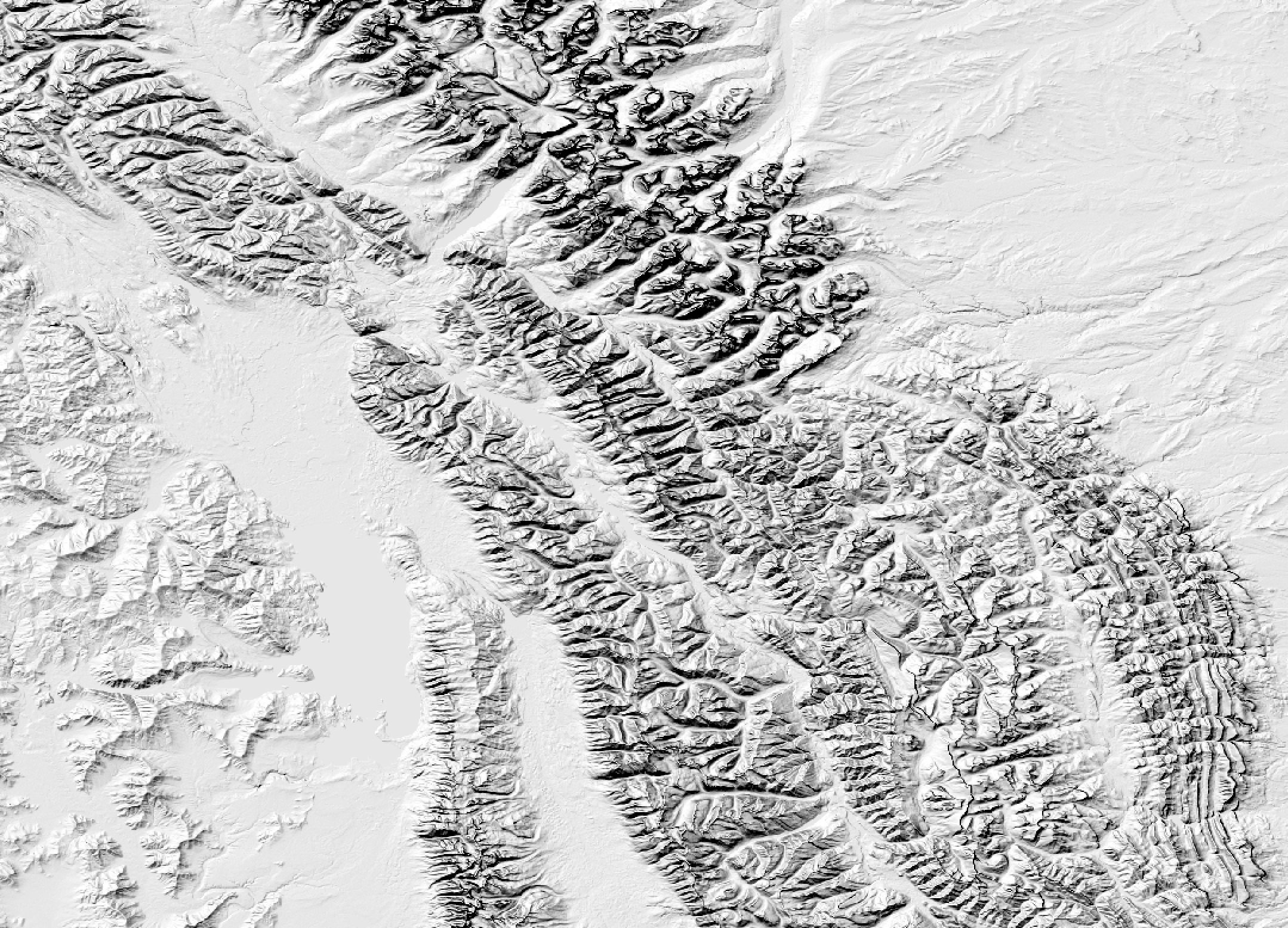Parameters:

FlagDescription
-i, --demInput raster DEM file
-o, --outputOutput raster file
--altitudeIllumination source altitude in degrees
--zfactorOptional multiplier for when the vertical and horizontal units are not the same
--full_modeOptional flag indicating whether to use full 360-degrees of illumination sources

Python function:

``````wbt.multidirectional_hillshade(
dem,
output,
altitude=45.0,
zfactor=None,
full_mode=False,
callback=default_callback
)
``````

Command-line Interface:

``````>>./whitebox_tools -r=MultidirectionalHillshade -v ^
--wd="/path/to/data/" -i=DEM.tif -o=output.tif ^
--altitude=30.0
``````

Source code on GitHub

Author: Dr. John Lindsay

Created: 19/07/2020

# MultiscaleElevationPercentile

This tool calculates the most elevation percentile (EP) across a range of spatial scales. EP is a measure of local topographic position (LTP) and expresses the vertical position for a digital elevation model (DEM) grid cell (z0) as the percentile of the elevation distribution within the filter window, such that:

EP = counti∈C(zi > z0) x (100 / nC)

where z0 is the elevation of the window's center grid cell, zi is the elevation of cell i contained within the neighboring set C, and nC is the number of grid cells contained within the window.

EP is unsigned and expressed as a percentage, bound between 0% and 100%. This tool outputs two rasters, the multiscale EP magnitude (`--out_mag`) and the scale at which the most extreme EP value occurs (`--out_scale`). The magnitude raster is the most extreme EP value (i.e. the furthest from 50%) for each grid cell encountered within the tested scales of EP.

Quantile-based estimates (e.g., the median and interquartile range) are often used in nonparametric statistics to provide data variability estimates without assuming the distribution is normal. Thus, EP is largely unaffected by irregularly shaped elevation frequency distributions or by outliers in the DEM, resulting in a highly robust metric of LTP. In fact, elevation distributions within small to medium sized neighborhoods often exhibit skewed, multimodal, and non-Gaussian distributions, where the occurrence of elevation errors can often result in distribution outliers. Thus, based on these statistical characteristics, EP is considered one of the most robust representation of LTP.

The algorithm implemented by this tool uses the relatively efficient running-histogram filtering algorithm of Huang et al. (1979). Because most DEMs contain floating point data, elevation values must be rounded to be binned. The `--sig_digits` parameter is used to determine the level of precision preserved during this binning process. The algorithm is parallelized to further aid with computational efficiency.

Experience with multiscale EP has shown that it is highly variable at shorter scales and changes more gradually at broader scales. Therefore, a nonlinear scale sampling interval is used by this tool to ensure that the scale sampling density is higher for short scale ranges and coarser at longer tested scales, such that:

ri = rL + [step × (i - rL)]p

Where ri is the filter radius for step i and p is the nonlinear scaling factor (`--step_nonlinearity`) and a step size (`--step`) of step.

References:

Newman, D. R., Lindsay, J. B., and Cockburn, J. M. H. (2018). Evaluating metrics of local topographic position for multiscale geomorphometric analysis. Geomorphology, 312, 40-50.

Huang, T., Yang, G.J.T.G.Y. and Tang, G., 1979. A fast two-dimensional median filtering algorithm. IEEE Transactions on Acoustics, Speech, and Signal Processing, 27(1), pp.13-18.

See Also: `ElevationPercentile`, MaxElevationDeviation, MaxDifferenceFromMean

Parameters:

FlagDescription
-i, --demInput raster DEM file
--out_magOutput raster roughness magnitude file
--out_scaleOutput raster roughness scale file
--sig_digitsNumber of significant digits
--min_scaleMinimum search neighbourhood radius in grid cells
--stepStep size as any positive non-zero integer
--num_stepsNumber of steps
--step_nonlinearityStep nonlinearity factor (1.0-2.0 is typical)

Python function:

``````wbt.multiscale_elevation_percentile(
dem,
out_mag,
out_scale,
sig_digits=3,
min_scale=4,
step=1,
num_steps=10,
step_nonlinearity=1.0,
callback=default_callback
)
``````

Command-line Interface:

``````>>./whitebox_tools -r=MultiscaleElevationPercentile -v ^
--wd="/path/to/data/" --dem=DEM.tif --out_mag=roughness_mag.tif ^
--out_scale=roughness_scale.tif --min_scale=1 --step=5 ^
--num_steps=100 --step_nonlinearity=1.5
``````

Source code on GitHub

Author: Dr. John Lindsay

Created: 22/12/2019

# MultiscaleRoughness

Calculates surface roughness over a range of spatial scales.

Parameters:

FlagDescription
-i, --demInput raster DEM file
--out_magOutput raster roughness magnitude file
--out_scaleOutput raster roughness scale file
--min_scaleMinimum search neighbourhood radius in grid cells
--max_scaleMaximum search neighbourhood radius in grid cells
--stepStep size as any positive non-zero integer

Python function:

``````wbt.multiscale_roughness(
dem,
out_mag,
out_scale,
max_scale,
min_scale=1,
step=1,
callback=default_callback
)
``````

Command-line Interface:

``````>>./whitebox_tools -r=MultiscaleRoughness -v ^
--wd="/path/to/data/" --dem=DEM.tif --out_mag=roughness_mag.tif ^
--out_scale=roughness_scale.tif --min_scale=1 --max_scale=1000 ^
--step=5
``````

Source code on GitHub

Author: Dr. John Lindsay

Created: 26/02/2018

# MultiscaleRoughnessSignature

Calculates the surface roughness for points over a range of spatial scales.

Parameters:

FlagDescription
-i, --demInput raster DEM file
--pointsInput vector points file
-o, --outputOutput HTML file
--min_scaleMinimum search neighbourhood radius in grid cells
--max_scaleMaximum search neighbourhood radius in grid cells
--stepStep size as any positive non-zero integer

Python function:

``````wbt.multiscale_roughness_signature(
dem,
points,
output,
max_scale,
min_scale=1,
step=1,
callback=default_callback
)
``````

Command-line Interface:

``````>>./whitebox_tools -r=MultiscaleRoughnessSignature -v ^
--wd="/path/to/data/" --dem=DEM.tif --points=sites.shp ^
--output=roughness.html --min_scale=1 --max_scale=1000 ^
--step=5
``````

Source code on GitHub

Author: Dr. John Lindsay

Created: 27/02/2018

# MultiscaleStdDevNormals

This tool can be used to map the spatial pattern of maximum spherical standard deviation (σs max; `--out_mag`), as well as the scale at which maximum spherical standard deviation occurs (rmax; `--out_scale`), for each grid cell in an input DEM (`--dem`). This serves as a multi-scale measure of surface roughness, or topographic complexity. The spherical standard deviation (σs) is a measure of the angular spread among n unit vectors and is defined as:

σs = √[-2ln(R / N)] × 180 / π

Where R is the resultant vector length and is derived from the sum of the x, y, and z components of each of the n normals contained within a filter kernel, which designates a tested spatial scale. Each unit vector is a 3-dimensional measure of the surface orientation and slope at each grid cell center. The maximum spherical standard deviation is:

σs max=max{σs(r):r=rL...rU},

Experience with roughness scale signatures has shown that σs max is highly variable at shorter scales and changes more gradually at broader scales. Therefore, a nonlinear scale sampling interval is used by this tool to ensure that the scale sampling density is higher for short scale ranges and coarser at longer tested scales, such that:

ri = rL + [step × (i - rL)]p

Where ri is the filter radius for step i and p is the nonlinear scaling factor (`--step_nonlinearity`) and a step size (`--step`) of step.

Use the SphericalStdDevOfNormals tool if you need to calculate σs for a single scale.

Reference:

JB Lindsay, DR Newman, and A Francioni. 2019 Scale-Optimized Surface Roughness for Topographic Analysis. Geosciences, 9(322) doi: 10.3390/geosciences9070322.

Parameters:

FlagDescription
-i, --demInput raster DEM file
--out_magOutput raster roughness magnitude file
--out_scaleOutput raster roughness scale file
--min_scaleMinimum search neighbourhood radius in grid cells
--stepStep size as any positive non-zero integer
--num_stepsNumber of steps
--step_nonlinearityStep nonlinearity factor (1.0-2.0 is typical)

Python function:

``````wbt.multiscale_std_dev_normals(
dem,
out_mag,
out_scale,
min_scale=1,
step=1,
num_steps=10,
step_nonlinearity=1.0,
callback=default_callback
)
``````

Command-line Interface:

``````>>./whitebox_tools -r=MultiscaleStdDevNormals -v ^
--wd="/path/to/data/" --dem=DEM.tif --out_mag=roughness_mag.tif ^
--out_scale=roughness_scale.tif --min_scale=1 --step=5 ^
--num_steps=100 --step_nonlinearity=1.5
``````

Source code on GitHub

Author: Dr. John Lindsay

Created: 05/06/2019

# MultiscaleStdDevNormalsSignature

Calculates the surface roughness for points over a range of spatial scales.

Parameters:

FlagDescription
-i, --demInput raster DEM file
--pointsInput vector points file
-o, --outputOutput HTML file
--min_scaleMinimum search neighbourhood radius in grid cells
--stepStep size as any positive non-zero integer
--num_stepsNumber of steps
--step_nonlinearityStep nonlinearity factor (1.0-2.0 is typical)

Python function:

``````wbt.multiscale_std_dev_normals_signature(
dem,
points,
output,
min_scale=1,
step=1,
num_steps=10,
step_nonlinearity=1.0,
callback=default_callback
)
``````

Command-line Interface:

``````>>./whitebox_tools -r=MultiscaleStdDevNormalsSignature -v ^
--wd="/path/to/data/" --dem=DEM.tif --points=sites.shp ^
--output=roughness.html --min_scale=1 --step=5 --num_steps=100 ^
--step_nonlinearity=1.5
``````

Source code on GitHub

Author: Dr. John Lindsay

Created: 20/06/2019

# MultiscaleTopographicPositionImage

This tool creates a multiscale topographic position (MTP) image (see here for an example) from three DEVmax rasters of differing spatial scale ranges. Specifically, MultiscaleTopographicPositionImage takes three DEVmax magnitude rasters, created using the MaxElevationDeviation tool, as inputs. The three inputs should correspond to the elevation deviations in the local (`--local`), meso (`--meso`), and broad (`--broad`) scale ranges and will be forced into the blue, green, and red colour components of the colour composite output (`--output`) raster. The image lightness value (`--lightness`) controls the overall brightness of the output image, as depending on the topography and scale ranges, these images can appear relatively dark. Higher values result in brighter, more colourful output images.

The output images can take some training to interpret correctly and a detailed explanation can be found in Lindsay et al. (2015). Sites within the landscape that occupy prominent topographic positions, either low-lying or elevated, will be apparent by their bright colouring in the MTP image. Those that are coloured more strongly in the blue are promient at the local scale range; locations that are more strongly green coloured are promient at the meso scale; and bright reds in the MTP image are associated with broad-scale landscape prominence. Of course, combination colours are also possible when topography is elevated or low-lying across multiple scale ranges. For example, a yellow area would indicated a site of prominent topographic position across the meso and broadest scale ranges.

Reference:

Lindsay J, Cockburn J, Russell H. 2015. An integral image approach to performing multi-scale topographic position analysis. Geomorphology, 245: 51-61.

Parameters:

FlagDescription
--localInput local-scale topographic position (DEVmax) raster file
--mesoInput meso-scale topographic position (DEVmax) raster file
-o, --outputOutput raster file
--lightnessImage lightness value (default is 1.2)

Python function:

``````wbt.multiscale_topographic_position_image(
local,
meso,
output,
lightness=1.2,
callback=default_callback
)
``````

Command-line Interface:

``````>>./whitebox_tools -r=MultiscaleTopographicPositionImage -v ^
--wd="/path/to/data/" --local=DEV_local.tif --meso=DEV_meso.tif ^
``````

Source code on GitHub

Author: Dr. John Lindsay

Created: 19/07/2017

# NumDownslopeNeighbours

This tool calculates the number of downslope neighbours of each grid cell in a raster digital elevation model (DEM). The user must specify the name of the input DEM (`--dem`) and the output (`--output`) raster name. The tool examines the eight neighbouring cells for each grid cell in a the DEM and counts the number of neighbours with an elevation less than the centre cell of the 3 x 3 window. The output image can therefore have values raning from 0 to 8. A raster grid cell with eight downslope neighbours is a peak and a cell with zero downslope neighbours is a pit. This tool can be used with the NumUpslopeNeighbours tool to assess the degree of local flow divergence/convergence.

Parameters:

FlagDescription
-i, --demInput raster DEM file
-o, --outputOutput raster file

Python function:

``````wbt.num_downslope_neighbours(
dem,
output,
callback=default_callback
)
``````

Command-line Interface:

``````>>./whitebox_tools -r=NumDownslopeNeighbours -v ^
--wd="/path/to/data/" -i=DEM.tif -o=output.tif
``````

Source code on GitHub

Author: Dr. John Lindsay

Created: 22/06/2017

# NumUpslopeNeighbours

This tool calculates the number of upslope neighbours of each grid cell in a raster digital elevation model (DEM). The user must specify the name of the input DEM (`--dem`) and the output (`--output`) raster name. The tool examines the eight neighbouring cells for each grid cell in a the DEM and counts the number of neighbours with an elevation less than the centre cell of the 3 x 3 window. The output raster can therefore have values ranging from 0 to 8, although in a DEM that has been hydrologically conditioned (i.e. depressions and flats removed), the values of the output will not exceed seven. This tool can be used with the NumDownslopeNeighbours tool to assess the degree of local flow divergence/convergence.

Parameters:

FlagDescription
-i, --demInput raster DEM file
-o, --outputOutput raster file

Python function:

``````wbt.num_upslope_neighbours(
dem,
output,
callback=default_callback
)
``````

Command-line Interface:

``````>>./whitebox_tools -r=NumUpslopeNeighbours -v ^
--wd="/path/to/data/" -i=DEM.tif -o=output.tif
``````

Source code on GitHub

Author: Dr. John Lindsay

Created: 22/06/2017

# Openness

This tool calculates the Yokoyama et al. (2002) topographic openness index from an input DEM (`--input`). Openness has two viewer perspectives, which correspond with positive and negative openness outputs (`--pos_output` and `--neg_output`). Positive values, expressing openness above the surface, are high for convex forms, whereas negative values describe this attribute below the surface and are high for concave forms. Openness is an angular value that is an average of the horizon angle in the eight cardinal directions to a maximum search distance (`--dist`), measured in grid cells. Openness rasters are best visualized using a greyscale palette.

Positive Openness: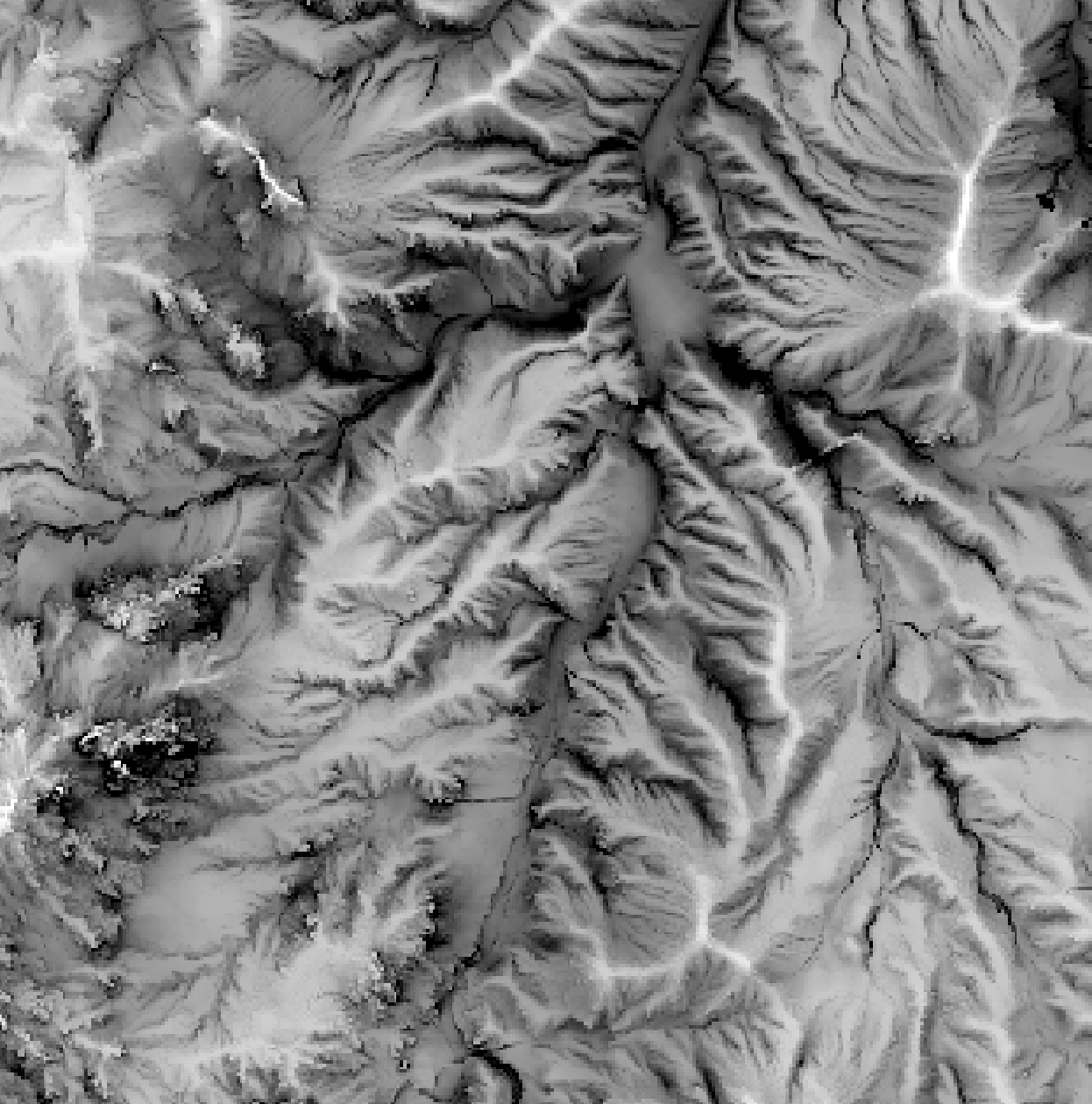Negative Openness: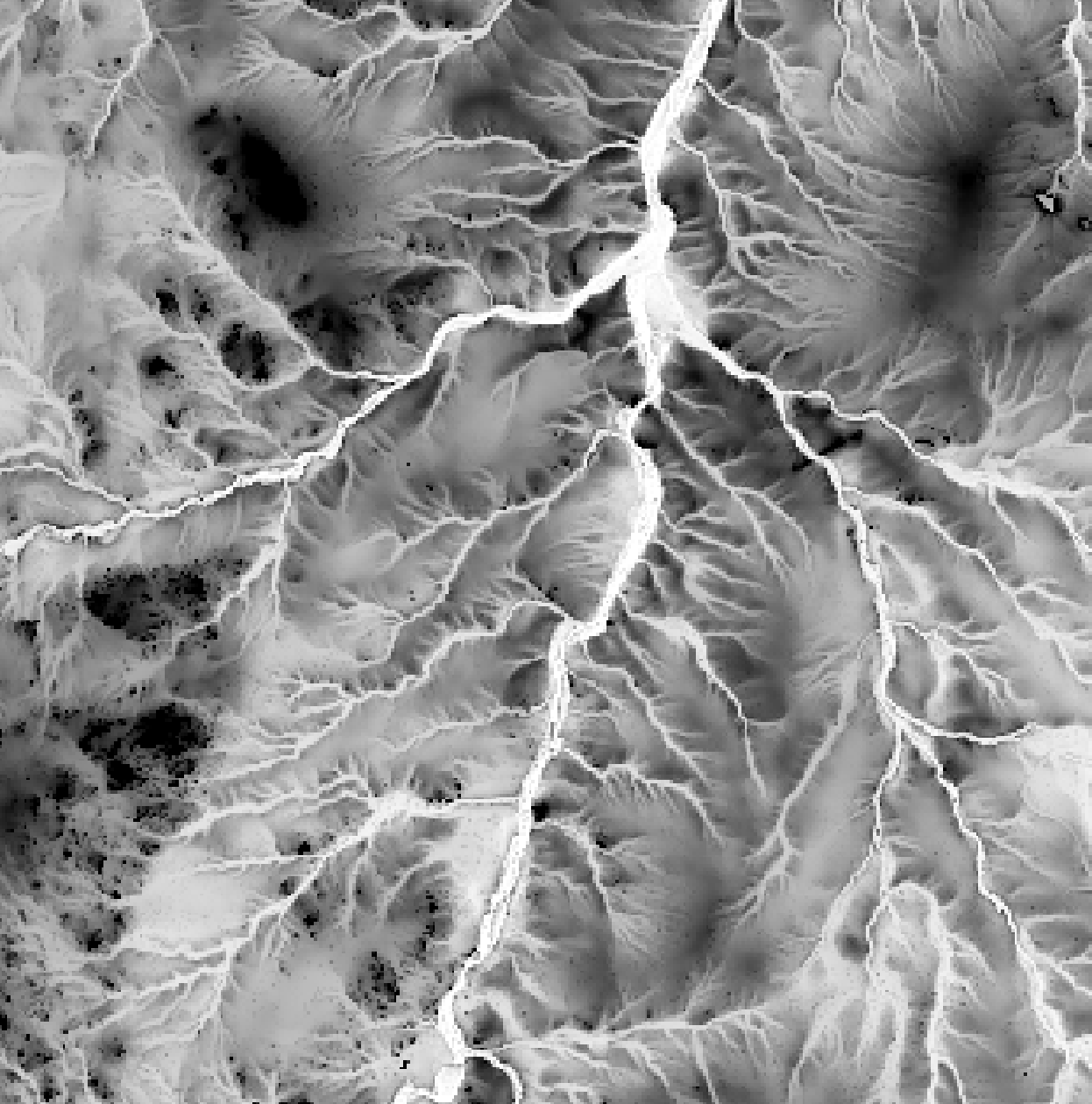References:

Yokoyama, R., Shirasawa, M., & Pike, R. J. (2002). Visualizing topography by openness: a new application of image processing to digital elevation models. Photogrammetric engineering and remote sensing, 68(3), 257-266.

Parameters:

FlagDescription
-i, --inputName of the input raster image file
--pos_outputName of the positive openness output raster file
--neg_outputName of the negative openness output raster file
--distSearch distance, in grid cells

Python function:

``````wbt.openness(
i,
pos_output,
neg_output,
dist=20,
callback=default_callback
)
``````

Command-line Interface:

``````>> ./whitebox_tools -r=Openness --input=DEM.tif ^
--pos_output=positive_openness.tif ^
--neg_output=negative_openness.tif --dist=500
``````

Source code is unavailable due to proprietary license.

Author: Whitebox Geospatial Inc. (c)

Created: 30/03/2021

# PennockLandformClass

Tool can be used to perform a simple landform classification based on measures of slope gradient and curvature derived from a user-specified digital elevation model (DEM). The classification scheme is based on the method proposed by Pennock, Zebarth, and DeJong (1987). The scheme divides a landscape into seven element types, including: convergent footslopes (CFS), divergent footslopes (DFS), convergent shoulders (CSH), divergent shoulders (DSH), convergent backslopes (CBS), divergent backslopes (DBS), and level terrain (L). The output raster image will record each of these base element types as:

Element TypeCode
CFS1
DFS2
CSH3
DSH4
CBS5
DBS6
L7

The definition of each of the elements, based on the original Pennock et al. (1987) paper, is as follows:

Concave ( -0.10)High >3.0Concave 0.0CFS
Concave ( -0.10)High >3.0Convex >0.0DFS
Convex (>0.10)High >3.0Concave 0.0CSH
Convex (>0.10)High >3.0Convex >0.0DSH
Linear (-0.10...0.10)High >3.0Concave 0.0CBS
Linear (-0.10...0.10)High >3.0Convex >0.0DBS
--Low 3.0--L

Where PROFILE is profile curvature, GRADIENT is the slope gradient, and PLAN is the plan curvature. Note that these values are likely landscape and data specific and can be adjusted by the user. Landscape classification schemes that are based on terrain attributes are highly sensitive to short-range topographic variability (i.e. roughness) and can benefit from pre-processing the DEM with a smoothing filter to reduce the effect of surface roughness and emphasize the longer-range topographic signal. The FeaturePreservingSmoothing tool offers excellent performance in smoothing DEMs without removing the sharpness of breaks-in-slope.

Reference:

Pennock, D.J., Zebarth, B.J., and DeJong, E. (1987) Landform classification and soil distribution in hummocky terrain, Saskatchewan, Canada. Geoderma, 40: 297-315.

Parameters:

FlagDescription
-i, --demInput raster DEM file
-o, --outputOutput raster file
--slopeSlope threshold value, in degrees (default is 3.0)
--profProfile curvature threshold value (default is 0.1)
--planPlan curvature threshold value (default is 0.0)
--zfactorOptional multiplier for when the vertical and horizontal units are not the same

Python function:

``````wbt.pennock_landform_class(
dem,
output,
slope=3.0,
prof=0.1,
plan=0.0,
zfactor=None,
callback=default_callback
)
``````

Command-line Interface:

``````>>./whitebox_tools -r=PennockLandformClass -v ^
--wd="/path/to/data/" --dem=DEM.tif -o=output.tif --slope=3.0 ^
--prof=0.1 --plan=0.0
``````

Source code on GitHub

Author: Dr. John Lindsay

Created: 12/07/2017

# PercentElevRange

Percent elevation range (PER) is a measure of local topographic position (LTP). It expresses the vertical position for a digital elevation model (DEM) grid cell (z0) as the percentage of the elevation range within the neighbourhood filter window, such that:

PER = z0 / (zmax - zmin) x 100

where z0 is the elevation of the window's center grid cell, zmax is the maximum neighbouring elevation, and zmin is the minimum neighbouring elevation.

Neighbourhood size, or filter size, is specified in the x and y dimensions using the `--filterx` and `--filtery`flags. These dimensions should be odd, positive integer values (e.g. 3, 5, 7, 9, etc.).

Compared with ElevPercentile and DevFromMeanElev, PER is a less robust measure of LTP that is susceptible to outliers in neighbouring elevations (e.g. the presence of off-terrain objects in the DEM).

References:

Newman, D. R., Lindsay, J. B., and Cockburn, J. M. H. (2018). Evaluating metrics of local topographic position for multiscale geomorphometric analysis. Geomorphology, 312, 40-50.

Parameters:

FlagDescription
-i, --input, --demInput raster DEM file
-o, --outputOutput raster file
--filterxSize of the filter kernel in the x-direction
--filterySize of the filter kernel in the y-direction

Python function:

``````wbt.percent_elev_range(
dem,
output,
filterx=3,
filtery=3,
callback=default_callback
)
``````

Command-line Interface:

``````>>./whitebox_tools -r=PercentElevRange -v ^
--wd="/path/to/data/" -i=DEM.tif -o=output.tif --filter=25
``````

Source code on GitHub

Author: Dr. John Lindsay

Created: 25/06/2017

# PlanCurvature

This tool calculates the plan curvature (i.e. contour curvature), or the rate of change in aspect along a contour line, from a digital elevation model (DEM). Curvature is the second derivative of the topographic surface defined by a DEM. Plan curvature characterizes the degree of flow convergence or divergence within the landscape (Gallant and Wilson, 2000). The user must specify the name of the input DEM (`--dem`) and the output raster image. WhiteboxTools reports curvature in degrees multiplied by 100 for easier interpretation. The Z conversion factor (`--zfactor`) is only important when the vertical and horizontal units are not the same in the DEM. When this is the case, the algorithm will multiply each elevation in the DEM by the Z Conversion Factor. If the DEM is in the geographic coordinate system (latitude and longitude), the following equation is used:

zfactor = 1.0 / (111320.0 x cos(mid_lat))

where `mid_lat` is the latitude of the centre of the raster, in radians.

The algorithm uses the same formula for the calculation of plan curvature as Gallant and Wilson (2000). Plan curvature is negative for diverging flow along ridges and positive for convergent areas, e.g. along valley bottoms.

Reference:

Gallant, J. C., and J. P. Wilson, 2000, Primary topographic attributes, in Terrain Analysis: Principles and Applications, edited by J. P. Wilson and J. C. Gallant pp. 51-86, John Wiley, Hoboken, N.J.

Parameters:

FlagDescription
-i, --demInput raster DEM file
-o, --outputOutput raster file
--zfactorOptional multiplier for when the vertical and horizontal units are not the same

Python function:

``````wbt.plan_curvature(
dem,
output,
zfactor=None,
callback=default_callback
)
``````

Command-line Interface:

``````>>./whitebox_tools -r=PlanCurvature -v --wd="/path/to/data/" ^
--dem=DEM.tif -o=output.tif
``````

Source code on GitHub

Author: Dr. John Lindsay

Created: 01/06/2017

# Profile

This tool can be used to plot the data profile, along a set of one or more vector lines (`--lines`), in an input (`--surface`) digital elevation model (DEM), or other surface model. The data profile plots surface height (y-axis) against distance along profile (x-axis). The tool outputs an interactive SVG line graph embedded in an HTML document (`--output`). If the vector lines file contains multiple line features, the output plot will contain each of the input profiles.

If you want to extract the longitudinal profile of a river, use the LongProfile tool instead.

Parameters:

FlagDescription
--linesInput vector line file
--surfaceInput raster surface file
-o, --outputOutput HTML file

Python function:

``````wbt.profile(
lines,
surface,
output,
callback=default_callback
)
``````

Command-line Interface:

``````>>./whitebox_tools -r=Profile -v --wd="/path/to/data/" ^
--lines=profile.shp --surface=dem.tif -o=profile.html
``````

Source code on GitHub

Author: Dr. John Lindsay

Created: 21/02/2018

# ProfileCurvature

This tool calculates the profile curvature, or the rate of change in slope along a flow line, from a digital elevation model (DEM). Curvature is the second derivative of the topographic surface defined by a DEM. Profile curvature characterizes the degree of downslope acceleration or deceleration within the landscape (Gallant and Wilson, 2000). The user must specify the name of the input DEM (`--dem`) and the output raster image. WhiteboxTools reports curvature in degrees multiplied by 100 for easier interpretation because curvature values are typically very small. The Z conversion factor (`--zfactor`) is only important when the vertical and horizontal units are not the same in the DEM. When this is the case, the algorithm will multiply each elevation in the DEM by the Z Conversion Factor. If the DEM is in the geographic coordinate system (latitude and longitude), the following equation is used:

zfactor = 1.0 / (111320.0 x cos(mid_lat))

where `mid_lat` is the latitude of the centre of the raster, in radians.

The algorithm uses the same formula for the calculation of plan curvature as Gallant and Wilson (2000). Profile curvature is negative for slope increasing downhill (convex flow profile, typical of upper slopes) and positive for slope decreasing downhill (concave, typical of lower slopes).

Reference:

Gallant, J. C., and J. P. Wilson, 2000, Primary topographic attributes, in Terrain Analysis: Principles and Applications, edited by J. P. Wilson and J. C. Gallant pp. 51-86, John Wiley, Hoboken, N.J.

Parameters:

FlagDescription
-i, --demInput raster DEM file
-o, --outputOutput raster file
--zfactorOptional multiplier for when the vertical and horizontal units are not the same

Python function:

``````wbt.profile_curvature(
dem,
output,
zfactor=None,
callback=default_callback
)
``````

Command-line Interface:

``````>>./whitebox_tools -r=ProfileCurvature -v ^
--wd="/path/to/data/" --dem=DEM.tif -o=output.tif
``````

Source code on GitHub

Author: Dr. John Lindsay

Created: 22/062017

# RelativeAspect

This tool creates a new raster in which each grid cell is assigned the terrain aspect relative to a user-specified direction (`--azimuth`). Relative terrain aspect is the angular distance (measured in degrees) between the land-surface aspect and the assumed regional wind azimuth (Bohner and Antonic, 2007). It is bound between 0-degrees (windward direction) and 180-degrees (leeward direction). Relative terrain aspect is the simplest of the measures of topographic exposure to wind, taking into account terrain orientation only and neglecting the influences of topographic shadowing by distant landforms and the deflection of wind by topography.

The user must specify the name of a digital elevation model (DEM) (`--dem`) and an azimuth (i.e. a wind direction). The Z Conversion Factor (`--zfactor`) is only important when the vertical and horizontal units are not the same in the DEM. When this is the case, the algorithm will multiply each elevation in the DEM by the Z Conversion Factor.

Reference:

Böhner, J., and Antonić, O. (2009). Land-surface parameters specific to topo-climatology. Developments in Soil Science, 33, 195-226.

Parameters:

FlagDescription
-i, --demInput raster DEM file
-o, --outputOutput raster file
--azimuthIllumination source azimuth
--zfactorOptional multiplier for when the vertical and horizontal units are not the same

Python function:

``````wbt.relative_aspect(
dem,
output,
azimuth=0.0,
zfactor=None,
callback=default_callback
)
``````

Command-line Interface:

``````>>./whitebox_tools -r=RelativeAspect -v --wd="/path/to/data/" ^
--dem=DEM.tif -o=output.tif --azimuth=180.0
``````

Source code on GitHub

Author: Dr. John Lindsay

Created: 17/06/2017

# RelativeTopographicPosition

Relative topographic position (RTP) is an index of local topographic position (i.e. how elevated or low-lying a site is relative to its surroundings) and is a modification of percent elevation range (PER; PercentElevRange) and accounts for the elevation distribution. Rather than positioning the central cell's elevation solely between the filter extrema, RTP is a piece-wise function that positions the central elevation relative to the minimum (zmin), mean (μ), and maximum values (zmax), within a local neighbourhood of a user-specified size (`--filterx`, `--filtery`), such that:

RTP = (z0 − μ) / (μ − zmin), if z0 < μ

OR

RTP = (z0 − μ) / (zmax - μ), if z0 >= μ 

The resulting index is bound by the interval [−1, 1], where the sign indicates if the cell is above or below than the filter mean. Although RTP uses the mean to define two linear functions, the reliance on the filter extrema is expected to result in sensitivity to outliers. Furthermore, the use of the mean implies assumptions of unimodal and symmetrical elevation distribution.

In many cases, Elevation Percentile (ElevPercentile) and deviation from mean elevation (DevFromMeanElev) provide more suitable and robust measures of relative topographic position.

Reference:

Newman, D. R., Lindsay, J. B., and Cockburn, J. M. H. (2018). Evaluating metrics of local topographic position for multiscale geomorphometric analysis. Geomorphology, 312, 40-50.

Parameters:

FlagDescription
-i, --demInput raster DEM file
-o, --outputOutput raster file
--filterxSize of the filter kernel in the x-direction
--filterySize of the filter kernel in the y-direction

Python function:

``````wbt.relative_topographic_position(
dem,
output,
filterx=11,
filtery=11,
callback=default_callback
)
``````

Command-line Interface:

``````>>./whitebox_tools -r=RelativeTopographicPosition -v ^
--wd="/path/to/data/" --dem=DEM.tif -o=output.tif ^
--filter=25
``````

Source code on GitHub

Author: Dr. John Lindsay

Created: 06/06/2017

# RemoveOffTerrainObjects

This tool can be used to create a bare-earth DEM from a fine-resolution digital surface model. The tool is typically applied to LiDAR DEMs which frequently contain numerous off-terrain objects (OTOs) such as buildings, trees and other vegetation, cars, fences and other anthropogenic objects. The algorithm works by finding and removing steep-sided peaks within the DEM. All peaks within a sub-grid, with a dimension of the user-specified maximum OTO size (`--filter`), in pixels, are identified and removed. Each of the edge cells of the peaks are then examined to see if they have a slope that is less than the user-specified minimum OTO edge slope (`--slope`) and a back-filling procedure is used. This ensures that OTOs are distinguished from natural topographic features such as hills. The DEM is preprocessed using a white top-hat transform, such that elevations are normalized for the underlying ground surface.

Note that this tool is appropriate to apply to rasterized LiDAR DEMs. Use the LidarGroundPointFilter tool to remove or classify OTOs within a LiDAR point-cloud.

Reference:

J.B. Lindsay (2018) A new method for the removal of off-terrain objects from LiDAR-derived raster surface models. Available online, DOI: 10.13140/RG.2.2.21226.62401

Parameters:

FlagDescription
-i, --input, --demInput raster DEM file
-o, --outputOutput raster file
--filterFilter size (cells)
--slopeSlope threshold value

Python function:

``````wbt.remove_off_terrain_objects(
dem,
output,
filter=11,
slope=15.0,
callback=default_callback
)
``````

Command-line Interface:

``````>>./whitebox_tools -r=RemoveOffTerrainObjects -v ^
--wd="/path/to/data/" --dem=DEM.tif -o=bare_earth_DEM.tif ^
--filter=25 --slope=10.0
``````

Source code on GitHub

Author: Dr. John Lindsay

Created: 06/06/2017

# RuggednessIndex

The terrain ruggedness index (TRI) is a measure of local topographic relief. The TRI calculates the root-mean-square-deviation (RMSD) for each grid cell in a digital elevation model (DEM), calculating the residuals (i.e. elevation differences) between a grid cell and its eight neighbours. Notice that, unlike the output of this tool, the original Riley et al. (1999) TRI did not normalize for the number of cells in the local window (i.e. it is a root-square-deviation only). However, using the mean has the advantage of allowing for the varying number of neighbouring cells along the grid edges and in areas bordering NoData cells. This modification does however imply that the ouput of this tool cannot be directly compared with the index ranges of level to extremely rugged terrain provided in Riley et al. (1999)

Reference:

Riley, S. J., DeGloria, S. D., and Elliot, R. (1999). Index that quantifies topographic heterogeneity. Intermountain Journal of Sciences, 5(1-4), 23-27.

Parameters:

FlagDescription
-i, --demInput raster DEM file
-o, --outputOutput raster file
--zfactorOptional multiplier for when the vertical and horizontal units are not the same

Python function:

``````wbt.ruggedness_index(
dem,
output,
zfactor=None,
callback=default_callback
)
``````

Command-line Interface:

``````>>./whitebox_tools -r=RuggednessIndex -v ^
--wd="/path/to/data/" --dem=DEM.tif -o=output.tif
``````

Source code on GitHub

Author: Dr. John Lindsay

Created: 22/06/2017

# SedimentTransportIndex

This tool calculates the sediment transport index, or sometimes, length-slope (LS) factor, based on input specific contributing area (As, i.e. the upslope contributing area per unit contour length; `--sca`) and slope gradient (β, measured in degrees; `--slope`) rasters. Moore et al. (1991) state that the physical potential for sheet and rill erosion in upland catchments can be evaluated by the product R K LS, a component of the Universal Soil Loss Equation (USLE), where R is a rainfall and runoff erosivity factor, K is a soil erodibility factor, and LS is the length-slope factor that accounts for the effects of topography on erosion. To predict erosion at a point in the landscape the LS factor can be written as:

LS = (n + 1)(As / 22.13)n(sin(β) / 0.0896)m

where n = 0.4 (`--sca_exponent`) and m = 1.3 (`--slope_exponent`) in its original formulation.

This index is derived from unit stream-power theory and is sometimes used in place of the length-slope factor in the revised universal soil loss equation (RUSLE) for slope lengths less than 100 m and slope less than 14 degrees. Like many hydrological land-surface parameters SedimentTransportIndex assumes that contributing area is directly related to discharge. Notice that As must not be log-transformed prior to being used; As is commonly log-transformed to enhance visualization of the data. Also, As can be derived using any of the available flow accumulation tools, alghough better results usually result from application of multiple-flow direction algorithms such as DInfFlowAccumulation and FD8FlowAccumulation. The slope raster can be created from the base digital elevation model (DEM) using the Slope tool. The input images must have the same grid dimensions.

Reference:

Moore, I. D., Grayson, R. B., and Ladson, A. R. (1991). Digital terrain modelling: a review of hydrological, geomorphological, and biological applications. Hydrological processes, 5(1), 3-30.

Parameters:

FlagDescription
--scaInput raster specific contributing area (SCA) file
--slopeInput raster slope file
-o, --outputOutput raster file
--sca_exponentSCA exponent value
--slope_exponentSlope exponent value

Python function:

``````wbt.sediment_transport_index(
sca,
slope,
output,
sca_exponent=0.4,
slope_exponent=1.3,
callback=default_callback
)
``````

Command-line Interface:

``````>>./whitebox_tools -r=SedimentTransportIndex -v ^
--wd="/path/to/data/" --sca='flow_accum.tif' ^
--slope='slope.tif' -o=output.tif --sca_exponent=0.5 ^
--slope_exponent=1.0
``````

Source code on GitHub

Author: Dr. John Lindsay

Created: 02/07/2017

This tool creates an interactive animated GIF of shadows based on an input digital surface model (DSM). The shadow model is based on the modelled positions of the sun throughout a user-specified date (`--date`) sampling at a regular interval (`--interval`), in minutes. Similar to the TimeInDaylight tool, this tool uses calculated horizon angle (HorizonAngle) values and a solar position model to determine which grid cells are located in shadow areas due to distant obsticles. The calculation of horizon angle, requires the user input a maximum search distance parameter (`--max_dist`).

The output (`--output`) of this tool is an HTML file, containing the interactive GIF animation. Users are able to zoom and pan around the displayed DEV animation. The DSM may be rendered in one of several available palettes (`--palette`) suitable for visualization topography. The user must also specify the image height (`--height`) in the output file, the time delay (`--delay`, in milliseconds) used in the GIF animation, and an optional label (`--label`), which will appear in the upper lefthand corner. Note that the output is simply HTML, CSS, javascript code, and a GIF file, which can be readily embedded in other documents.

Users should be aware that the outut GIF can be very large in size, depending on the size of the input DEM file. To reduce the file size of the output, it may be desirable to coarsen the input DEM resolution using image resampling (Resample).

The following is an example of what the output of this tool looks like. Click the image for an interactive example.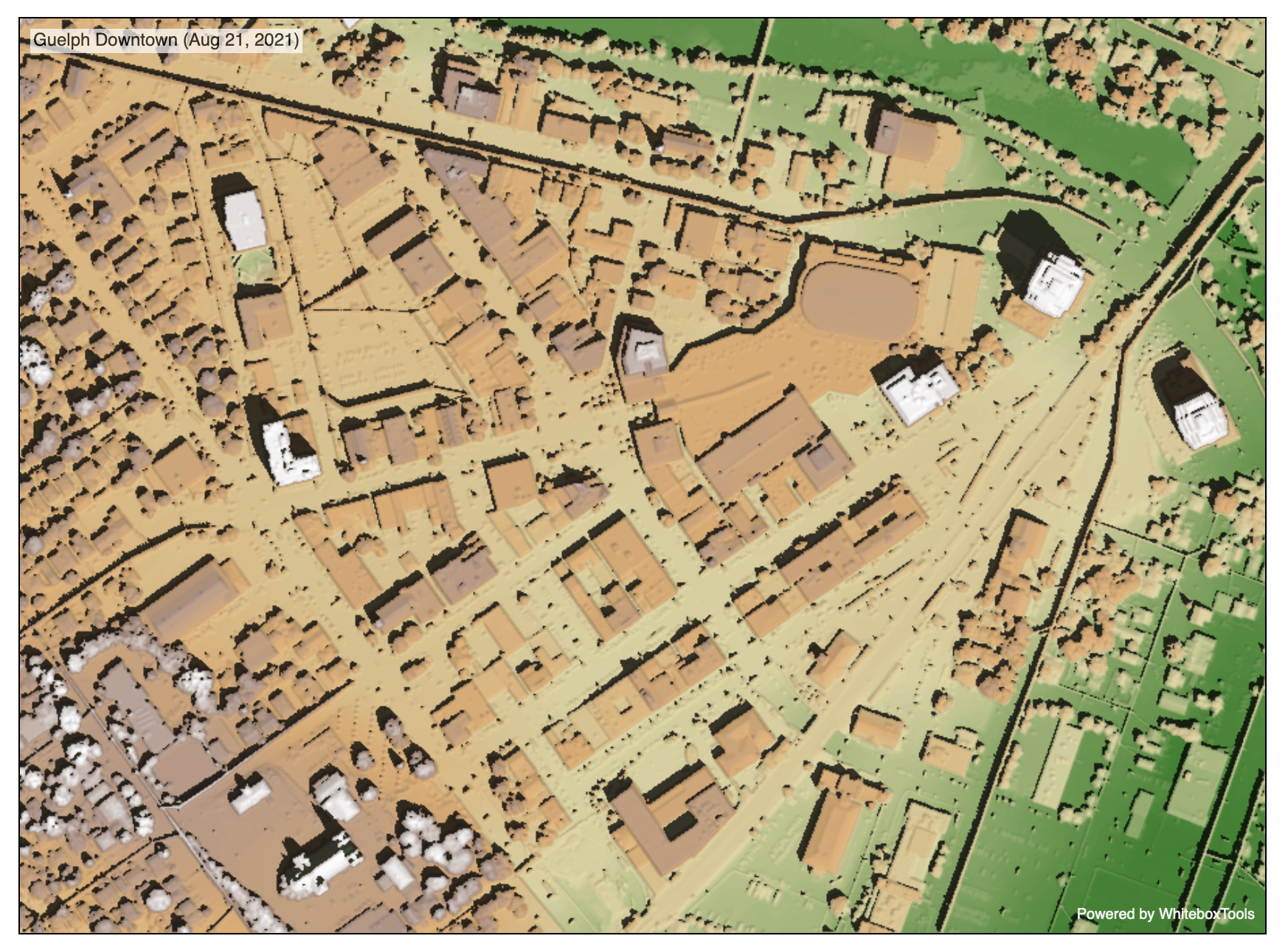Parameters:

FlagDescription
-i, --inputName of the input digital surface model (DSM) raster file
-p, --paletteDSM image palette; options are 'atlas', 'high_relief', 'arid', 'soft', 'muted', 'light_quant', 'purple', 'viridi', 'gn_yl', 'pi_y_g', 'bl_yl_rd', 'deep', and 'none'
-o, --outputName of the output HTML file (*.html)
--max_distOptional maximum search distance. Minimum value is 5 x cell size
-d, --dateDate in format DD/MM/YYYY
--intervalTime interval, in minutes (1-60)
--locationLocation, defined as Lat/Long/UTC-offset (e.g. 43.5448/-80.2482/-4)
-h, --heightImage height, in pixels
--delayGIF time delay in milliseconds
-l, --labelLabel text (leave blank for none)

Python function:

``````wbt.shadow_animation(
i,
output,
palette="atlas",
max_dist="",
date="21/06/2021",
interval=15,
location="43.5448/-80.2482/-4",
height=600,
delay=250,
label="",
callback=default_callback
)
``````

Command-line Interface:

``````>> ./whitebox_tools -r=ShadowAnimation -i=dsm.tif ^
--date='21/06/2021' --interval=20 --location='43.55/ -80.25/ ^
``````

Source code is unavailable due to proprietary license.

Author: Whitebox Geospatial Inc. (c)

Created: 01/05/2021

# Slope

This tool calculates slope gradient (i.e. slope steepness in degrees, radians, or percent) for each grid cell in an input digital elevation model (DEM). The user must specify the name of the input DEM (`--dem`) and the output raster image. The Z conversion factor is only important when the vertical and horizontal units are not the same in the DEM. When this is the case, the algorithm will multiply each elevation in the DEM by the Z conversion factor. If the DEM is in the geographic coordinate system (latitude and longitude), the following equation is used:

zfactor = 1.0 / (111320.0 x cos(mid_lat))

where `mid_lat` is the latitude of the centre of the raster, in radians.

The tool uses Horn's (1981) 3rd-order finite difference method to estimate slope. Given the following clock-type grid cell numbering scheme (Gallant and Wilson, 2000),

| 7 | 8 | 1 |
| 6 | 9 | 2 |
| 5 | 4 | 3 |

slope = arctan(fx2 + fy2)0.5

where,

fx = (z3 - z5 + 2(z2 - z6) + z1 - z7) / 8 * Δx

and,

fy = (z7 - z5 + 2(z8 - z4) + z1 - z3) / * Δy

Δx and Δy are the grid resolutions in the x and y direction respectively

Reference:

Gallant, J. C., and J. P. Wilson, 2000, Primary topographic attributes, in Terrain Analysis: Principles and Applications, edited by J. P. Wilson and J. C. Gallant pp. 51-86, John Wiley, Hoboken, N.J.

Parameters:

FlagDescription
-i, --demInput raster DEM file
-o, --outputOutput raster file
--zfactorOptional multiplier for when the vertical and horizontal units are not the same
--unitsUnits of output raster; options include 'degrees', 'radians', 'percent'

Python function:

``````wbt.slope(
dem,
output,
zfactor=None,
units="degrees",
callback=default_callback
)
``````

Command-line Interface:

``````>>./whitebox_tools -r=Slope -v --wd="/path/to/data/" ^
``````

Source code on GitHub

Author: Dr. John Lindsay

Created: 22/06/2017

# SlopeVsElevationPlot

This tool can be used to create a slope versus average elevation plot for one or more digital elevation models (DEMs). Similar to a hypsometric analysis (HypsometricAnalysis), the slope-elevation relation can reveal the basic topographic character of a site. The output of this analysis is an HTML document (`--output`) that contains the slope-elevation chart. The tool can plot multiple slope-elevation analyses on the same chart by specifying multiple input DEM files (`--inputs`). Each input DEM can have an optional watershed in which the slope-elevation analysis is confined by specifying the optional `--watershed` flag. If multiple input DEMs are used, and a watershed is used to confine the analysis to a sub-area, there must be the same number of input raster watershed files as input DEM files. The order of the DEM and watershed files must the be same (i.e. the first DEM file must correspond to the first watershed file, the second DEM file to the second watershed file, etc.). Each watershed file may contain one or more watersheds, designated by unique identifiers.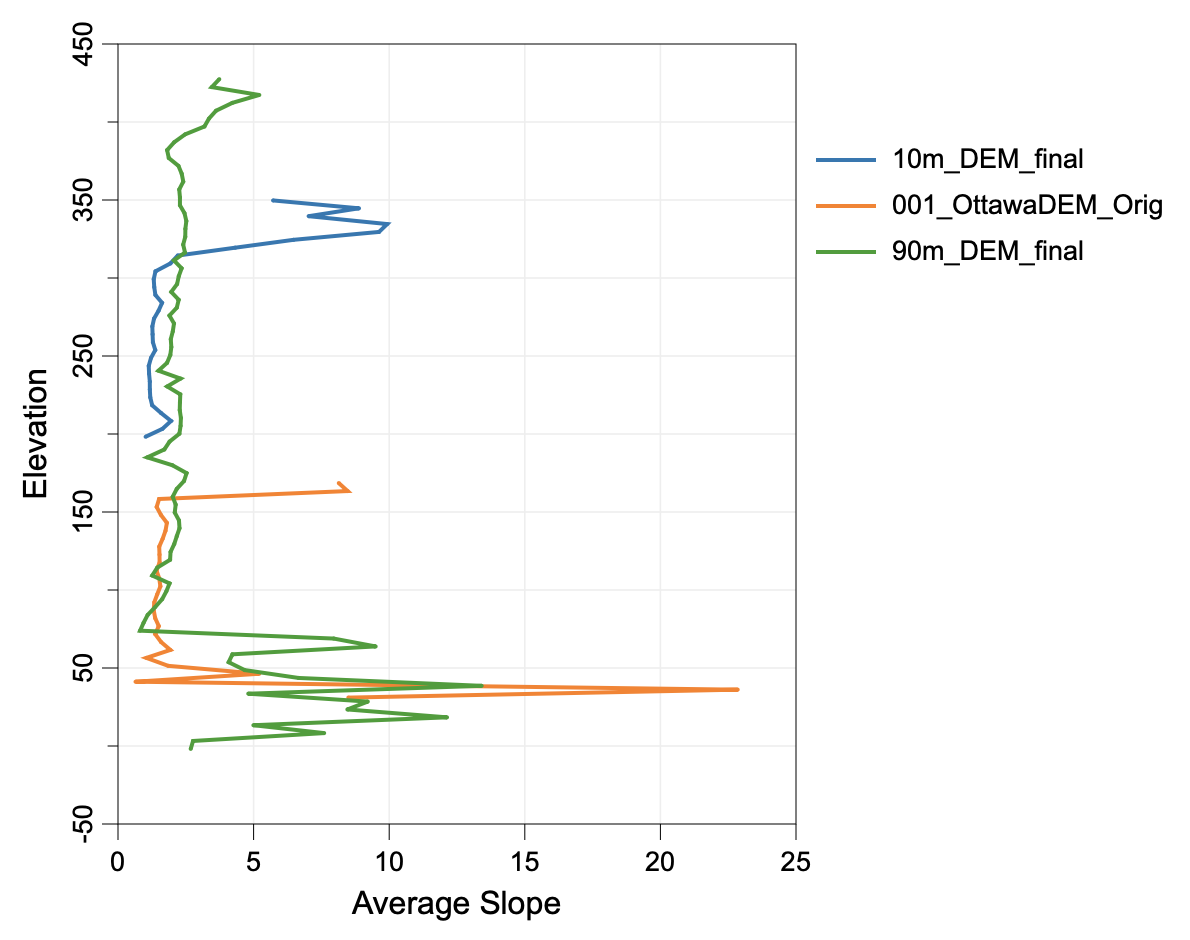Parameters:

FlagDescription
-i, --inputsInput DEM files
--watershedInput watershed files (optional)
-o, --outputOutput HTML file (default name will be based on input file if unspecified)

Python function:

``````wbt.slope_vs_elevation_plot(
inputs,
output,
watershed=None,
callback=default_callback
)
``````

Command-line Interface:

``````>>./whitebox_tools -r=SlopeVsElevationPlot -v ^
--wd="/path/to/data/" -i="DEM1.tif;DEM2.tif" ^
--watershed="ws1.tif;ws2.tif" -o=outfile.html
``````

Source code on GitHub

Author: Dr. John Lindsay

Created: 01/02/2018

# SmoothVegetationResidual

This tool can smooth the roughness due to residual vegetation cover in LiDAR digital elevation models (DEMs). Sometimes when LiDAR data are collected under heavy forest cover, particularly conifer species, the DEM will contain substantial roughness, even if it is interpolated using last-return points only. This tool can be used to reduce the roughness of the ground surface under these conditions. It works by identifying grid cells that possess deviation in mean elevation (DEV, DevFromMeanElev) values that are higher than a specified threshold value (`--dev_threshold`) for tested scales less than a specified threshold (`--scale_threshold`). DEV is measured for the input DEM (`--input`) using filter radii from 1 to a user-specified maximum (`--max_scale`). The identified grid cells are then masked out and their elevations are re-interpolated using the surrounding, non-masked values.

This method can work well under some conditions, and will further benefit from multiple passes of the tool, i.e. run the tool using one set of parameters and then use the output (`--output`) as the input for the second pass. Alternative approaches include use of the RemoveOffTerrainObjects tool, using low-pass filters such as the FeaturePreservingSmoothing tool, or, if the point-cloud source data are available, classifying the ground points using LidarGroundPointFilter and excluding non-ground points from the interpolation.

The following image shows an image of a DEM that is badly impacted by heavy forest cover, with obvious vegetation residual roughness.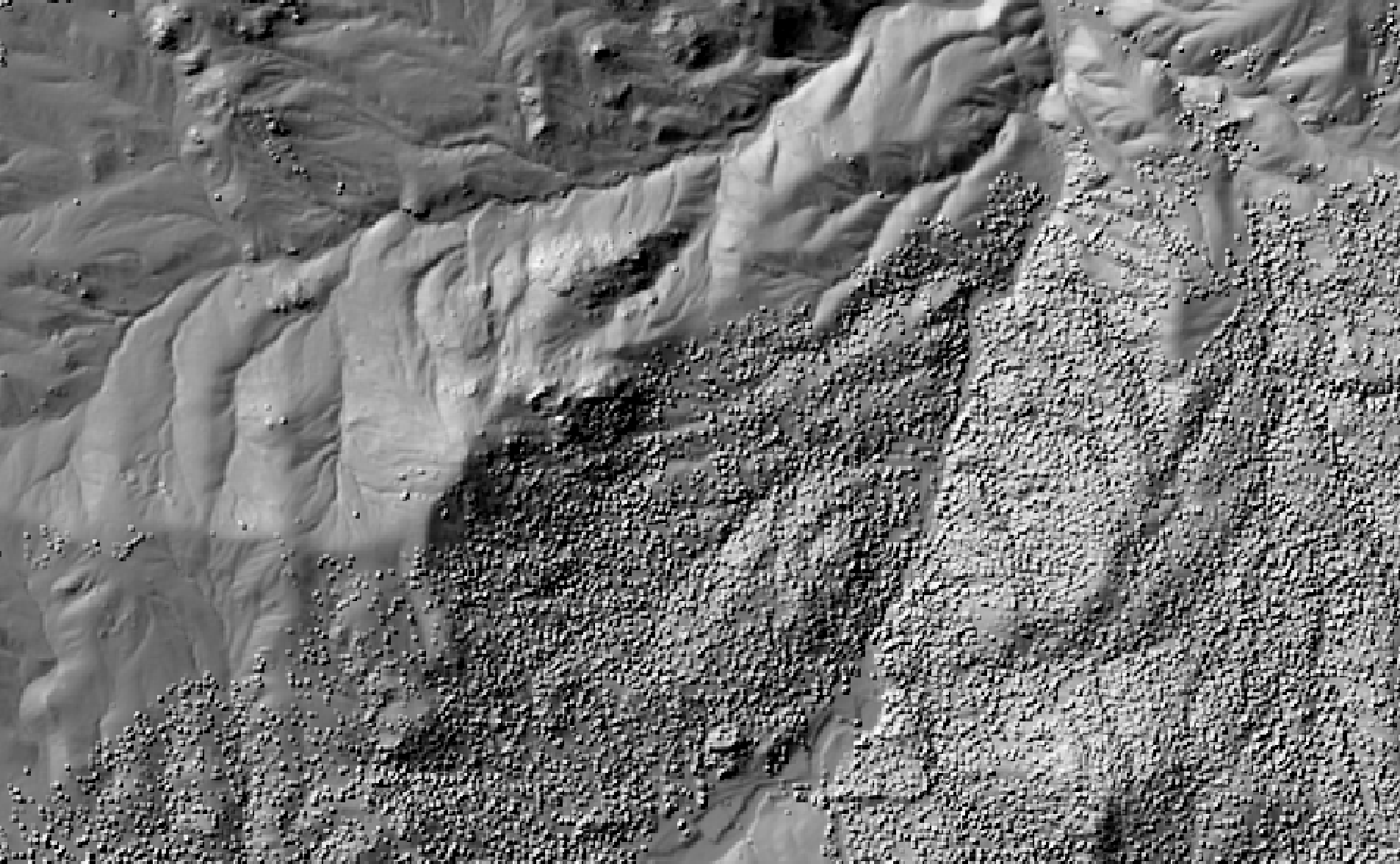This image shows the impact of two-passes of the SmoothVegetationResidual tool.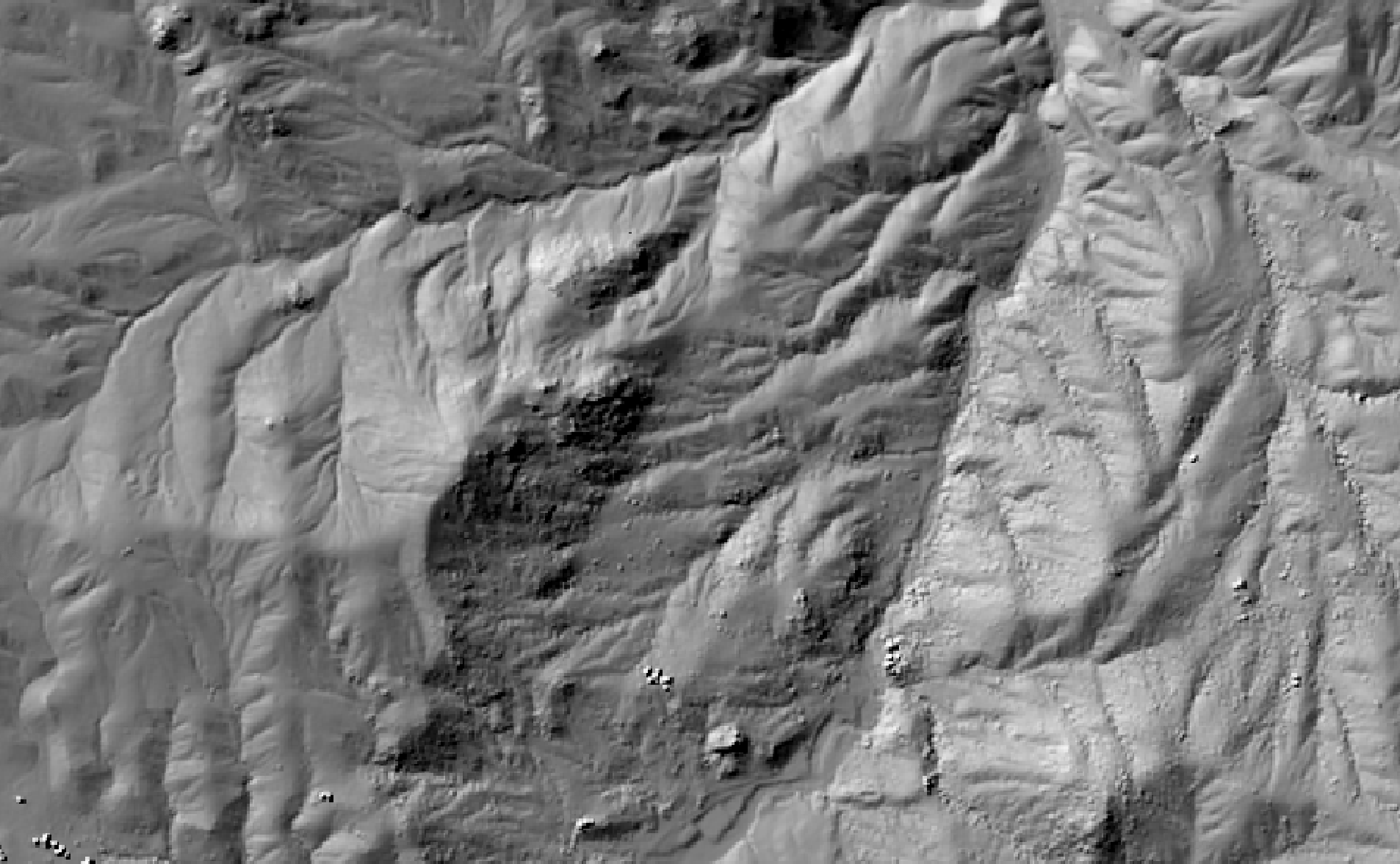See Also: RemoveOffTerrainObjects, FeaturePreservingSmoothing, LidarGroundPointFilter, DevFromMeanElev

Parameters:

FlagDescription
-i, --inputName of the input digital elevation model (DEM) raster file
-o, --outputName of the output raster file
--max_scaleMaximum search neighbourhood radius in grid cells
--dev_thresholdDEVmax Threshold
--scale_thresholdDEVmax scale threshold

Python function:

``````wbt.smooth_vegetation_residual(
i,
output,
max_scale=30,
dev_threshold=1.0,
scale_threshold=5,
callback=default_callback
)
``````

Command-line Interface:

``````-./whitebox_tools -r=SmoothVegetationResidual -i=DEM.tif ^
-o=smoothed_DEM.tif --max_scale=50 --dev_threshold=0.5 ^
--scale_threshold=8
``````

Source code is unavailable due to proprietary license.

Author: Whitebox Geospatial Inc. (c)

Created: 09/05/2021

# SphericalStdDevOfNormals

This tool can be used to calculate the spherical standard deviation of the distribution of surface normals for an input digital elevation model (DEM; `--dem`). This is a measure of the angular dispersion of the surface normal vectors within a local neighbourhood of a specified size (`--filter`). SphericalStdDevOfNormals is therefore a measure of surface shape complexity, texture, and roughness. The spherical standard deviation (s) is defined as:

s = √[-2ln(R / N)] × 180 / π

where R is the resultant vector length and N is the number of unit normal vectors within the local neighbourhood. s is measured in degrees and is zero for simple planes and increases infinitely with increasing surface complexity or roughness. Note that this formulation of the spherical standard deviation assumes an underlying wrapped normal distribution.

The local neighbourhood size (`--filter`) must be any odd integer equal to or greater than three. Grohmann et al. (2010) found that vector dispersion, a related measure of angular dispersion, increases monotonically with scale. This is the result of the angular dispersion measure integrating (accumulating) all of the surface variance of smaller scales up to the test scale. A more interesting scale relation can therefore be estimated by isolating the amount of surface complexity associated with specific scale ranges. That is, at large spatial scales, s should reflect the texture of large-scale landforms rather than the accumulated complexity at all smaller scales, including microtopographic roughness. As such, this tool normalizes the surface complexity of scales that are smaller than the filter size by applying Gaussian blur (with a standard deviation of one-third the filter size) to the DEM prior to calculating R. In this way, the resulting distribution is able to isolate and highlight the surface shape complexity associated with landscape features of a similar scale to that of the filter size.

This tool makes extensive use of integral images (i.e. summed-area tables) and parallel processing to ensure computational efficiency. It may, however, require substantial memory resources when applied to larger DEMs.

References:

Grohmann, C. H., Smith, M. J., & Riccomini, C. (2010). Multiscale analysis of topographic surface roughness in the Midland Valley, Scotland. IEEE Transactions on Geoscience and Remote Sensing, 49(4), 1200-1213.

Hodgson, M. E., and Gaile, G. L. (1999). A cartographic modeling approach for surface orientation-related applications. Photogrammetric Engineering and Remote Sensing, 65(1), 85-95.

Lindsay J. B., Newman* D. R., Francioni, A. 2019. Scale-optimized surface roughness for topographic analysis. Geosciences, 9(7) 322. DOI: 10.3390/geosciences9070322.

Parameters:

FlagDescription
-i, --demInput raster DEM file
--outputOutput raster file
--filterSize of the filter kernel

Python function:

``````wbt.spherical_std_dev_of_normals(
dem,
output,
filter=11,
callback=default_callback
)
``````

Command-line Interface:

``````>>./whitebox_tools -r=SphericalStdDevOfNormals -v ^
--wd="/path/to/data/" --dem=DEM.tif --output=roughness.tif ^
--filter=15
``````

Source code on GitHub

Author: Dr. John Lindsay

Created: 22/05/2019

# StandardDeviationOfSlope

Calculates the standard deviation of slope from an input DEM, a metric of roughness described by Grohmann et al., (2011).

Parameters:

FlagDescription
-i, --inputInput raster DEM file
-o, --outputOutput raster DEM file
--zfactorOptional multiplier for when the vertical and horizontal units are not the same
--filterxSize of the filter kernel in the x-direction
--filterySize of the filter kernel in the y-direction

Python function:

``````wbt.standard_deviation_of_slope(
i,
output,
zfactor=None,
filterx=11,
filtery=11,
callback=default_callback
)
``````

Command-line Interface:

``````>>./whitebox_tools -r=StandardDeviationOfSlope -v ^
--wd="/path/to/data/" --dem=DEM.tif -o=output.tif ^
--zfactor=1.0 --filterx=15 --filtery=15
``````

Source code on GitHub

Author: Anthony Francioni

Created: 26/05/2018

# StreamPowerIndex

This tool can be used to calculate the relative stream power (RSP) index. This index is directly related to the stream power if the assumption can be made that discharge is directly proportional to upslope contributing area (As; `--sca`). The index is calculated as:

RSP = Asp × tan(β)

where As is the specific catchment area (i.e. the upslope contributing area per unit contour length) estimated using one of the available flow accumulation algorithms; β is the local slope gradient in degrees (`--slope`); and, p (`--exponent`) is a user-defined exponent term that controls the location-specific relation between contributing area and discharge. Notice that As must not be log-transformed prior to being used; As is commonly log-transformed to enhance visualization of the data. The slope raster can be created from the base digital elevation model (DEM) using the Slope tool. The input images must have the same grid dimensions.

Reference:

Moore, I. D., Grayson, R. B., and Ladson, A. R. (1991). Digital terrain modelling: a review of hydrological, geomorphological, and biological applications. Hydrological processes, 5(1), 3-30.

Parameters:

FlagDescription
--scaInput raster specific contributing area (SCA) file
--slopeInput raster slope file
-o, --outputOutput raster file
--exponentSCA exponent value

Python function:

``````wbt.stream_power_index(
sca,
slope,
output,
exponent=1.0,
callback=default_callback
)
``````

Command-line Interface:

``````>>./whitebox_tools -r=StreamPowerIndex -v ^
--wd="/path/to/data/" --sca='flow_accum.tif' ^
--slope='slope.tif' -o=output.tif --exponent=1.1
``````

Source code on GitHub

Author: Dr. John Lindsay

Created: 02/07/2017

# SurfaceAreaRatio

This tool calculates the ratio between the surface area and planar area of grid cells within digital elevation models (DEMs). The tool uses the method of Jenness (2004) to estimate the surface area of a DEM grid cell based on the elevations contained within the 3 x 3 neighbourhood surrounding each cell. The surface area ratio has a lower bound of 1.0 for perfectly flat grid cells and is greater than 1.0 for other conditions. In particular, surface area ratio is a measure of neighbourhood surface shape complexity (texture) and elevation variability (local slope).

Reference:

Jenness, J. S. (2004). Calculating landscape surface area from digital elevation models. Wildlife Society Bulletin, 32(3), 829-839.

Parameters:

FlagDescription
-i, --demInput raster DEM file
-o, --outputOutput raster file

Python function:

``````wbt.surface_area_ratio(
dem,
output,
callback=default_callback
)
``````

Command-line Interface:

``````>>./whitebox_tools -r=SurfaceAreaRatio -v ^
--wd="/path/to/data/" --dem=DEM.tif -o=output.tif
``````

Source code on GitHub

Author: Dr. John Lindsay

Created: 23/01/2019

# TangentialCurvature

This tool calculates the tangential curvature, which is the curvature of an inclined plan perpendicular to both the direction of flow and the surface (Gallant and Wilson, 2000). Curvature is a second derivative of the topographic surface defined by a digital elevation model (DEM). The user must specify the name of the input DEM (`--dem`) and the output raster image (`--output`). The output reports curvature in degrees multiplied by 100 for easier interpretation, as curvature values are often very small. The Z Conversion Factor (`--zfactor`) is only important when the vertical and horizontal units are not the same in the DEM. When this is the case, the algorithm will multiply each elevation in the DEM by the Z Conversion Factor. If the DEM is in the geographic coordinate system (latitude and longitude), with XY units measured in degrees, an appropriate Z Conversion Factor is calculated internally based on site latitude.

Reference:

Gallant, J. C., and J. P. Wilson, 2000, Primary topographic attributes, in Terrain Analysis: Principles and Applications, edited by J. P. Wilson and J. C. Gallant pp. 51-86, John Wiley, Hoboken, N.J.

Parameters:

FlagDescription
-i, --demInput raster DEM file
-o, --outputOutput raster file
--zfactorOptional multiplier for when the vertical and horizontal units are not the same

Python function:

``````wbt.tangential_curvature(
dem,
output,
zfactor=None,
callback=default_callback
)
``````

Command-line Interface:

``````>>./whitebox_tools -r=TangentialCurvature -v ^
--wd="/path/to/data/" --dem=DEM.tif -o=output.tif
``````

Source code on GitHub

Author: Dr. John Lindsay

Created: 22/06/2017

# TimeInDaylight

This tool calculates the proportion of time a location is within daylight. That is, it calculates the proportion of time, during a user-defined time frame, that a grid cell in an input digital elevation model (`--dem`) is outside of an area of shadow cast by a local object. The input DEM should truly be a digital surface model (DSM) that contains significant off-terrain objects. Such a model, for example, could be created using the first-return points of a LiDAR data set, or using the LidarDigitalSurfaceModel tool.

The tool operates by calculating a solar almanac, which estimates the sun's position for the location, in latitude and longitude coordinate (`--lat`, `--long`), of the input DSM. The algorithm then calculates horizon angle (see HorizonAngle) rasters from the DSM based on the user-specified azimuth fraction (`--az_fraction`). For example, if an azimuth fraction of 15-degrees is specified, horizon angle rasters could be calculated for the solar azimuths 0, 15, 30, 45... In reality, horizon angle rasters are only calculated for azimuths for which the sun is above the horizon for some time during the tested time period. A horizon angle raster evaluates the vertical angle between each grid cell in a DSM and a distant obstacle (e.g. a mountain ridge, building, tree, etc.) that blocks the view along a specified direction. In calculating horizon angle, the user must specify the maximum search distance (`--max_dist`) beyond which the query for higher, more distant objects will cease. This parameter strongly impacts the performance of the tool, with larger values resulting in significantly longer run-times. Users are advised to set the `--max_dist` based on the maximum shadow length expected in an area. For example, in a relatively flat urban landscape, the tallest building will likely determine the longest shadow lengths. All grid cells for which the calculated solar positions throughout the time frame are higher than the cell's horizon angle are deemed to be illuminated during the time the sun is in the corresponding azimuth fraction.

By default, the tool calculates time-in-daylight for a time-frame spanning an entire year. That is, the solar almanac is calculated for each hour, at 10-second intervals, and for each day of the year. Users may alternatively restrict the time of year over which time-in-daylight is calculated by specifying a starting day (1-365; `--start_day`) and ending day (1-365; `--end_day`). Similarly, by specifying start time (`--start_time`) and end time (`--end_time`) parameters, the user is able to measure time-in-daylight for specific ranges of the day (e.g. for the morning or afternoon hours). These time parameters must be specified in 24-hour time (HH:MM:SS), e.g. 15:30:00. `sunrise` and `sunset` are also acceptable inputs for the start time and end time respectively. The timing of sunrise and sunset on each day in the tested time-frame will be determined using the solar almanac.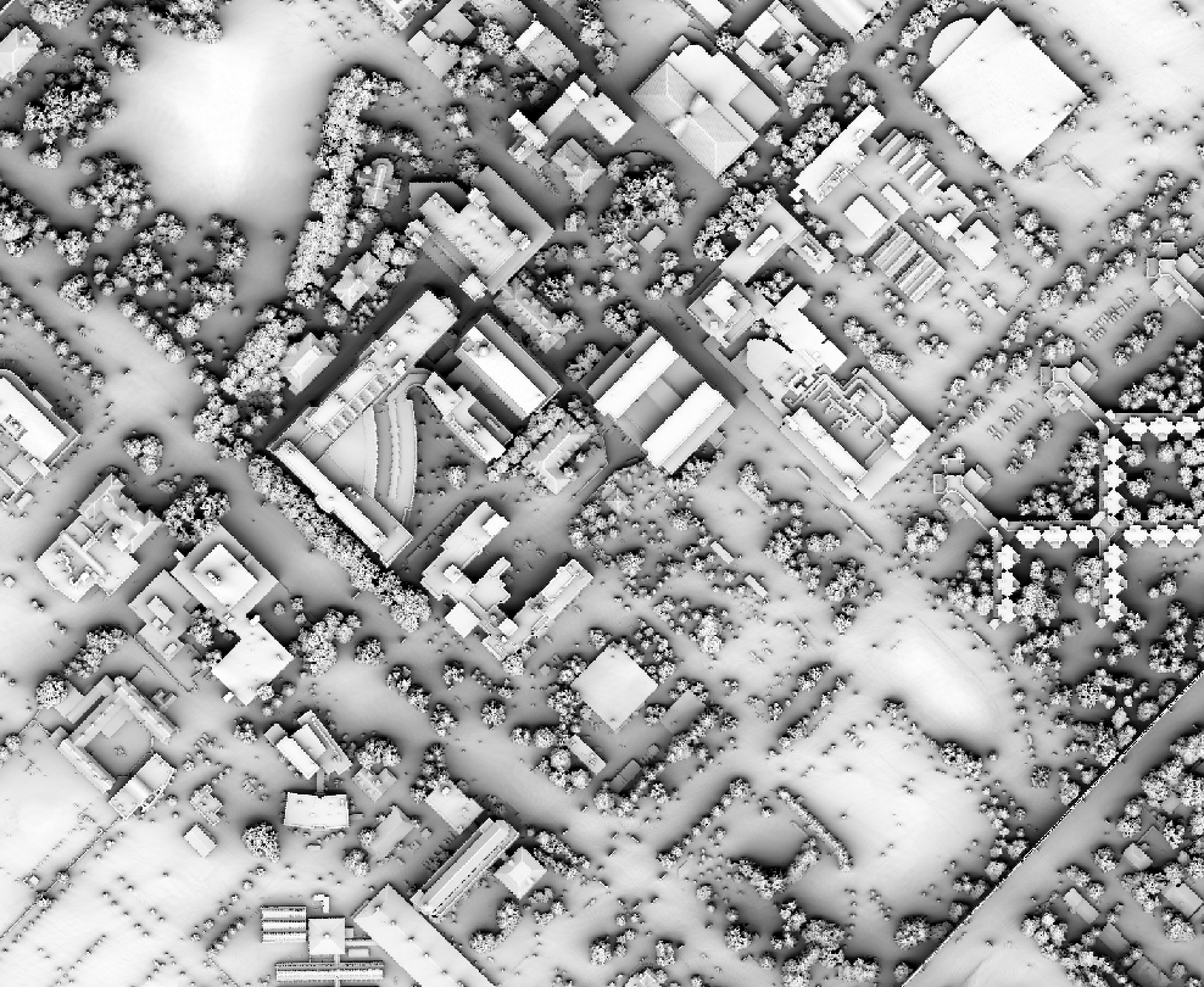Parameters:

FlagDescription
-i, --demInput raster DEM file
-o, --outputOutput raster file
--az_fractionAzimuth fraction in degrees
--max_distOptional maximum search distance. Minimum value is 5 x cell size
--latCentre point latitude
--longCentre point longitude
--utc_offsetUTC time offset, in hours (e.g. -04:00, +06:00)
--start_dayStart day of the year (1-365)
--end_dayEnd day of the year (1-365)
--start_timeStarting hour to track shadows (e.g. 5, 5:00, 05:00:00). Assumes 24-hour time: HH:MM:SS. 'sunrise' is also a valid time
--end_timeStarting hour to track shadows (e.g. 21, 21:00, 21:00:00). Assumes 24-hour time: HH:MM:SS. 'sunset' is also a valid time

Python function:

``````wbt.time_in_daylight(
dem,
output,
lat,
long,
az_fraction=10.0,
max_dist=100.0,
utc_offset="00:00",
start_day=1,
end_day=365,
start_time="00:00:00",
end_time="23:59:59",
callback=default_callback
)
``````

Command-line Interface:

``````>>./whitebox_tools -r=TimeInDaylight -v --wd="/path/to/data/" ^
-i='input.tif' -o=output.tif --az_fraction=15.0 ^
--max_dist=100.0 --lat=43.545 --long= -80.248
``````

Source code on GitHub

Author: Dr. John Lindsay

Created: 29/07/2020

# TopographicPositionAnimation

This tool creates an interactive animation that demonstrates the variation in deviation from mean elevation (DEV, DevFromMeanElev) as scale increases across a range for an input digital elevation model (`--input`). DEV is calculated as the difference between the elevation of each grid cell and the mean elevation of the centering local neighbourhood, normalized by standard deviation and is a measure of local topographic position. DEV is useful for highlighting locally prominent (either elevated or low-lying) locations within a landscape. Topographic position animations are extemely useful for interpreting landscape geomorphic structure across a range of scales.

The set of scales for which DEV is measured (using varying filter sizes) is determined by the three user-specified parameters, including `--min_scale`, `--num_steps`, and `--step_nonlinearity`. Experience with DEV scale signatures has shown that it is highly variable at shorter scales and changes more gradually at broader scales. Therefore, a nonlinear scale sampling interval is used by this tool to ensure that the scale sampling density is higher for short scale ranges and coarser at longer tested scales, such that:

ri = rL + (i - rL)p

Where ri is the filter radius for step i, rL is the lower range of filter sizes (`--min_scale`), and p is the nonlinear scaling factor (`--step_nonlinearity`).

The tool can be run in one of two modes: using regular DEV calculations, or using DEVmax (MaxElevationDeviation), a multiscale version of DEV that outputs the maximum absolute value of DEV encountered across a range of tested scales. Use the `--dev_max` flag to run the tool in DEVmax mode.

The output (`--output`) of this tool is an HTML file, containing the interactive GIF animation. Users are able to zoom and pan around the displayed DEV animation. The DEV images may be rendered in one of several available palettes (`--palette`) suitable for visualization DEV. The output DEV/DEVmax animation will also be hillshaded to further enchance topographic interpretation. The user must also specify the image height (`--height`) in the output file, the time delay (`--delay`, in milliseconds) used in the GIF animation, and an optional label (`--label`), which will appear in the upper lefthand corner. Note that the output is simply HTML, CSS, javascript code, and a GIF file, which can be readily embedded in other documents.

Users should be aware that the outut GIF can be very large in size, depending on the size of the input DEM file. To reduce the file size of the output, it may be desirable to coarsen the input DEM resolution using image resampling (Resample).

The following is an example of what the output of this tool looks like. Click the image for an interactive example.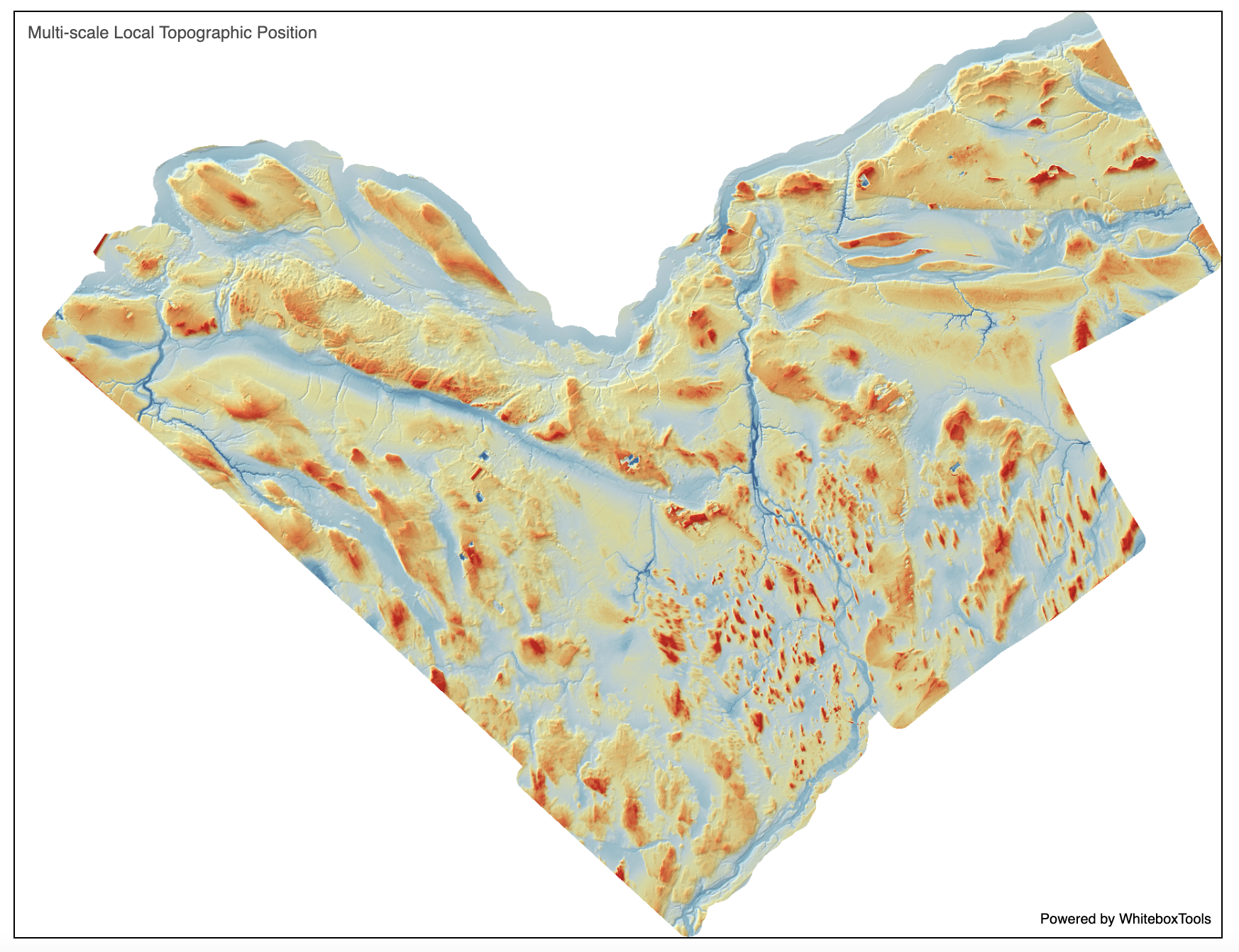Parameters:

FlagDescription
-i, --inputName of the input digital elevation model (DEM) raster file
-p, --paletteImage palette; options are 'bl_yl_rd', 'bl_w_rd', 'purple', 'gn_yl', 'pi_y_g', and 'viridi'
-o, --outputName of the output HTML file (*.html)
--min_scaleMinimum search neighbourhood radius in grid cells
--num_stepsNumber of steps
--step_nonlinearityStep nonlinearity factor (1.0-2.0 is typical)
--heightImage height, in pixels
--delayGIF time delay in milliseconds
-l, --labelLabel text (leave blank for none)
--dev_maxDo you want to use DEVmax instead of DEV for measuring local topographic position?

Python function:

``````wbt.topographic_position_animation(
i,
output,
palette="bl_yl_rd",
min_scale=1,
num_steps=100,
step_nonlinearity=1.5,
height=600,
delay=250,
label="",
dev_max=False,
callback=default_callback
)
``````

Command-line Interface:

``````>> ./whitebox_tools -r=TopographicPositionAnimation -i=dem.tif ^
-p='bl_w_rd' -o=DEV_animation.html --min_scale=3 ^
--num_steps=100 --step_nonlinearity=1.2 --height=620 ^
--delay=200 --label='DEVmax for Catfish Watershed' --dev_max
``````

Source code is unavailable due to proprietary license.

Author: Whitebox Geospatial Inc. (c)

Created: 06/05/2021

# TotalCurvature

This tool calculates the total curvature, which measures the curvature of the topographic surface rather than the curvature of a line across the surface in some direction (Gallant and Wilson, 2000). Total curvature can be positive or negative, with zero curvature indicating that the surface is either flat or the convexity in one direction is balanced by the concavity in another direction, as would occur at a saddle point. Curvature is a second derivative of the topographic surface defined by a digital elevation model (DEM). The user must specify the name of the input DEM (`--dem`) and the output raster image (`--output`). The output reports curvature in degrees multiplied by 100 for easier interpretation, as curvature values are often very small. The Z Conversion Factor (`--zfactor`) is only important when the vertical and horizontal units are not the same in the DEM. When this is the case, the algorithm will multiply each elevation in the DEM by the Z Conversion Factor. If the DEM is in the geographic coordinate system (latitude and longitude), with XY units measured in degrees, an appropriate Z Conversion Factor is calculated internally based on site latitude.

Reference:

Gallant, J. C., and J. P. Wilson, 2000, Primary topographic attributes, in Terrain Analysis: Principles and Applications, edited by J. P. Wilson and J. C. Gallant pp. 51-86, John Wiley, Hoboken, N.J.

Parameters:

FlagDescription
-i, --demInput raster DEM file
-o, --outputOutput raster file
--zfactorOptional multiplier for when the vertical and horizontal units are not the same

Python function:

``````wbt.total_curvature(
dem,
output,
zfactor=None,
callback=default_callback
)
``````

Command-line Interface:

``````>>./whitebox_tools -r=TotalCurvature -v --wd="/path/to/data/" ^
--dem=DEM.tif -o=output.tif
``````

Source code on GitHub

Author: Dr. John Lindsay

Created: 22/06/2017

# Viewshed

This tool can be used to calculate the viewshed (i.e. the visible area) from a location (i.e. viewing station) or group of locations based on the topography defined by an input digital elevation model (DEM). The user must specify the name of the input DEM (`--dem`), a viewing station input vector file (`--stations`), the output file name (`--output`), and the viewing height (`--height`). Viewing station locations are specified as points within an input shapefile. The output image indicates the number of stations visible from each grid cell. The viewing height is in the same units as the elevations of the DEM and represent a height above the ground elevation from which the viewshed is calculated.

Viewshed should be used when there are a relatively small number of target sites for which visibility needs to be assessed. If you need to assess general landscape visibility as a land-surface parameter, the VisibilityIndex tool should be used instead.

Viewshed analysis is a very computationally intensive task. Depending on the size of the input DEM grid and the number of viewing stations, this operation may take considerable time to complete. Also, this implementation of the viewshed algorithm does not account for the curvature of the Earth. This should be accounted for if viewsheds are being calculated over very extensive areas.

Parameters:

FlagDescription
--demInput raster DEM file
--stationsInput viewing station vector file
-o, --outputOutput raster file
--heightViewing station height, in z units

Python function:

``````wbt.viewshed(
dem,
stations,
output,
height=2.0,
callback=default_callback
)
``````

Command-line Interface:

``````>>./whitebox_tools -r=Viewshed -v --wd="/path/to/data/" ^
--dem='dem.tif' --stations='stations.shp' -o=output.tif ^
--height=10.0
``````

Source code on GitHub

Author: Dr. John Lindsay

Created: 10/01/2018

# VisibilityIndex

This tool can be used to calculate a measure of landscape visibility based on the topography of an input digital elevation model (DEM). The user must specify the name of the input DEM (`--dem`), the output file name (`--output`), the viewing height (`--height`), and a resolution factor (`--res_factor`). Viewsheds are calcuated for a subset of grid cells in the DEM based on the resolution factor. The visibility index value (0.0-1.0) indicates the proportion of tested stations (determined by the resolution factor) that each cell is visible from. The viewing height is in the same units as the elevations of the DEM and represent a height above the ground elevation. Each tested grid cell's viewshed will be calculated in parallel. However, visibility index is one of the most computationally intensive geomorphometric indices to calculate. Depending on the size of the input DEM grid and the resolution factor, this operation may take considerable time to complete. If the task is too long-running, it is advisable to raise the resolution factor. A resolution factor of 2 will skip every second row and every second column (effectively evaluating the viewsheds of a quarter of the DEM's grid cells). Increasing this value decreases the number of calculated viewshed but will result in a lower accuracy estimate of overall visibility. In addition to the high computational costs of this index, the tool also requires substantial memory resources to operate. Each of these limitations should be considered before running this tool on a particular data set. This tool is best to apply on computer systems with high core-counts and plenty of memory.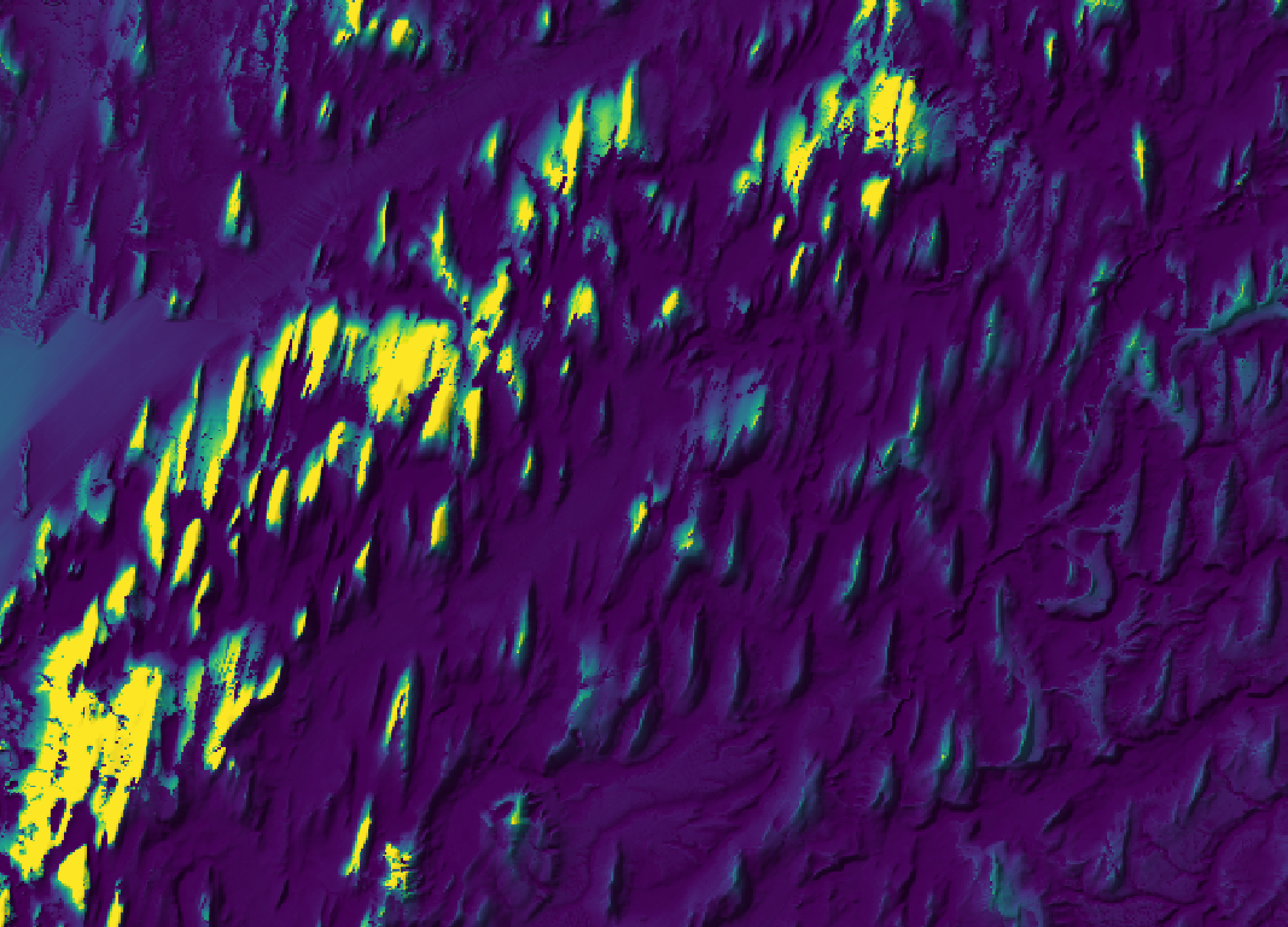Parameters:

FlagDescription
--demInput raster DEM file
-o, --outputOutput raster file
--heightViewing station height, in z units
--res_factorThe resolution factor determines the density of measured viewsheds

Python function:

``````wbt.visibility_index(
dem,
output,
height=2.0,
res_factor=2,
callback=default_callback
)
``````

Command-line Interface:

``````>>./whitebox_tools -r=VisibilityIndex -v ^
--wd="/path/to/data/" --dem=dem.tif -o=output.tif ^
--height=10.0 --res_factor=4
``````

Source code on GitHub

Author: Dr. John Lindsay

Created: 07/04/2018

# WetnessIndex

This tool can be used to calculate the topographic wetness index, commonly used in the TOPMODEL rainfall-runoff framework. The index describes the propensity for a site to be saturated to the surface given its contributing area and local slope characteristics. It is calculated as:

WI = Ln(As / tan(Slope))

Where `As` is the specific catchment area (i.e. the upslope contributing area per unit contour length) estimated using one of the available flow accumulation algorithms in the Hydrological Analysis toolbox. Notice that `As` must not be log-transformed prior to being used; log-transformation of `As` is a common practice when visualizing the data. The slope image should be measured in degrees and can be created from the base digital elevation model (DEM) using the Slope tool. Grid cells with a slope of zero will be assigned NoData in the output image to compensate for the fact that division by zero is infinity. These very flat sites likely coincide with the wettest parts of the landscape. The input images must have the same grid dimensions.

Grid cells possessing the NoData value in either of the input images are assigned NoData value in the output image. The output raster is of the float data type and continuous data scale.

Parameters:

FlagDescription
--scaInput raster specific contributing area (SCA) file
--slopeInput raster slope file (in degrees)
-o, --outputOutput raster file

Python function:

``````wbt.wetness_index(
sca,
slope,
output,
callback=default_callback
)
``````

Command-line Interface:

``````>>./whitebox_tools -r=WetnessIndex -v --wd="/path/to/data/" ^
--sca='flow_accum.tif' --slope='slope.tif' -o=output.tif
``````

Source code on GitHub

Author: Dr. John Lindsay

Created: 02/07/2017